# Cross-Validation with Python VIDEO

Cross-validation is a valuable tool for assessing a model’s ability to generalize. In the video below, we will look at how to use cross-validation with Python.

# Intro to Matplotlib with Python VIDEO

Matplotlib is a data visualization module used often in Python. In this video, we will go over some introductory basic commands. Doing so will allow anybody who wants to be able to make simple manipulations to their visualizations.

# Random Forest Regression with Python VIDEO

In the video below we will take a look at how to perform a random forest regression analysis with Python. Random forest is one of many tools that can be used in the field of data science to gain insights to help people.

# K Nearest Neighbor Classification with Python VIDEO

K nearest neighbor classification is another tool used in machine learning to predict what class an observation belongs to. In this video, we will learn how to implement this algorithm using Python.

# Naive Bayes with Python VIDEO

Naive Bayes is an algorithm that is commonly used with text classification. However, it can also be used for separating observations into multiple categories. In this video, we will look at a simple example of the use of Naive Bayes in Python.

# K-Nearest Neighbor Regression with Python VIDEO

K-Nearest neighbor is a great technique for dealing with data. In the video below, we will look at how to use this tool with Python.

# Support Vector Machines Regression with Python VIDEO

VIDEOIn this video, we will look at a simple example of SVM regression. In this context, regression involves predicting a continuous dependent variable. This is similar to the basic form of regression that is taught in an introduction to stats class

# Support Vector Machines Classification with Python VIDEO

Learn how to use support vector machines for classification using Python. This is another great algorithm for data science purposes.

# Linear Discriminant Analysis with Python VIDEO

Linear discriminant analysis is a tool that is used for classification. This tool is one of many that is employed in data science. In this video, we will look at an example of how to use this tool in Python for practical purposes.

# Factor Analysis with Python VIDEO

Factor analysis is a statistical technique used to reduce the number of dimensions in order to simplify additional analysis or confirm a construct. In this video, we will look at a very simple example of factor analysis along with a visualization.

# Natural Language Process and WordClouds with Python VIDEO

Natural language processing is a tool used in data science to modify texts in order to extract meaning. The video below will go through some basics of this processing. For an added bonus, there is also an example of making a word cloud.

# KMeans Clustering with Python VIDEO

Kmeans clustering is an unsupervised learning technique used to place date in various groups as determine by the algorithm. In this video, we will go step by step through the process of using this insight tool.

# Random Forest in Python VIDEO

Random forest is a machine learning algorithm that makes multiple decision trees in order to make the best decision. By making many trees you can avoid the mistake of overfitting to the data, which is a common weakness of decision trees.

# Decision Trees in Python VIDEO

Decision trees are common tool used in data science and machine learning. In the video below we will learn how to develop a simple decision tree using Python.

# Principal Component Analysis with Python VIDEO

Principal component analysis is a tool for reducing the number of variables in a dataset without losing too much information. This is a great way to summarize information or to simplify things for a more complex analysis. The video provides a simple example of how to do this.

# Data Visualization with Altair VIDEO

Python has a great library called that Altair that makes it really easy to make various data visualizations. The primary strength of this particular library is how easy it is to use and to also create interactive plots. The video below provides an introduction to using this innovative tool.# Visualizations with Altair

We are going to take a look at Altair which is a data visulization library for Python. What is unique abiut Altair compared to other packages experienced on this blog is that it allows for interactions.

The interactions can take place inside jupyter or they can be exported and loaded onto websites as we shall see. In the past, making interactions for website was often tught using a jacascript library such as d3.js. D3.js works but is cumbersome to work with for the avaerage non-coder. Altair solves this problem as Python is often seen as easier to work with compared to javascript.

Installing Altair

If Altair is not already install on your computer you can do so with the following code

``````pip install altair vega_datasets

OR

conda install -c conda-forge altair vega_datasets``````

Which one of the lines above you use will depend on the type of Python installation you have.

Goal

We are going to make some simple visualizations using the “Duncan” dataset from the pydataset library using Altair. If you do not have pydataset install on your ocmputer you can use the code listed above to install it. Simple replace “altair vega_datasets” with “pydataset.” Below is the initial code followed by the output

``````import pandas as pd
from pydataset import data
df=data("Duncan")

In the code above, we load pandas and import “data” from the “pydataset” library. Next, we load the “Duncan” dataset as the object “df”. Lastly, we use the .head() function to take a look at the dataset. You can see in the imagine above what variables are available.

Our first visualization is a simple bar graph. The code is below followed by the visualization.

``````import altair as alt
alt.Chart(df).mark_bar().encode(
x= "type",
y = "prestige"
)``````

In the code above we did the following,

1. Line one loads the altair library.
2. Line 2 uses several functions together to make the bar graph. .Chart(df) loads the data for the plot. .mark_bar() assigns the geomtric shape for the plot which in this case is bars. Lastly, the .encode() function contains the information for the variables that will be assigned to the x and y axes. In this case we are looking at job type and prestige.

The three dots in the upper right provide options for saving or editing the plot. We will learn more about saving plots later. In addition, Altair follows the grammar of graphics for creating plots. This has been discussed in another post but a summary of the components are below.

• Data
• Aesthetics
• Scale.
• Statistical transformation
• Geometric object
• Facets
• Coordinate system

We will not deal with all of these but we have dealt with the following

• Data as .Chart()
• Aesthetics and Geometric object as .mark_bar()
• coordinate system as .encode()

In our second example, we will make a scatterplot. The code and output are below.

``````alt.Chart(df).mark_circle().encode(
x= "education",
y = "prestige"
)``````

The code is mostly the same. We simple use .mark_circle() as to indicate the type of geometric object. For .encode() we made sure to use two continuous variables.

In the next plot, we add a categorical variable to the scatterplot by manipulating the color.

``````alt.Chart(df).mark_circle().encode(
x= "education",
y = "prestige",
color='type'
)``````

The only change is the addition of the “color”argument which is set to the categorical vareiable of “type.”

It is also possible to use bubbles to indicate size. In the plot below we can add the income varibale to the plot using bubbles.

``````alt.Chart(df).mark_circle().encode(
x= "education",
y = "prestige",
color='type',
size="income"
)``````

The latest argument that was added was the “size” argument which was used to map income to the plot.

You can also facet data by piping. The code below makes two plots and saving them as objects. Then you print both by typing the name of the objects while separated by the pipe symbol (|) which you can find above the enter key on your keyboard. Below you will find two different plots created through this piping process.

``````educationPlot=alt.Chart(df).mark_circle().encode(
x= "education",
y = "prestige",
color='type',
)
incomePlot=alt.Chart(df).mark_circle().encode(
x= "income",
y = "prestige",
color='type',
)
educationPlot | incomePlot``````

With this code you can make multiple plots. Simply keep adding pipes to make more plots.

Interaction and Saving Plots

It is also possible to move plots interactive. In the code below we add the command called tool tip. This allows us to add an additional variable called “income” to the chart. When the mouse hoovers over a data-point the income will display.

However, since we are in a browser right now this will not work unless w save the chart as an html file. The last line of code saves the plot as an html file and renders it using svg. We also remove the three dots in the upper left corner by adding the ‘actions’:False. Below is the code and the plot once the html was loaded to this blog.

``````interact_plot=alt.Chart(df).mark_circle().encode(
x= "education",
y = "prestige",
color='type',
tooltip=["income"]
)
interact_plot.save('interact_plot.html',embed_options={'renderer':'svg','actions':False})``````

I’ve made a lot of visuals in the past and never has it been this simple

Conclusion

Altair is another tool for visualizations. This may be the easiest way to make complex and interactive charts that I have seen. As such, this is a great way to achieve goals if visualizing data is something that needs to be done.# Random Forest Classification with Python

Random forest is a type of machine learning algorithm in which the algorithm makes multiple decision trees that may use different features and subsample to making as many trees as you specify. The trees then vote to determine the class of an example. This approach helps to deal with the high variance that is a problem with making only one decision tree.In this post, we will learn how to develop a random forest model in Python. We will use the cancer dataset from the pydataset module to classify whether a person status is censored or dead based on several independent variables. The steps we need to perform to complete this task are defined below

1. Data preparation
2. Model development and evaluation

Data Preparation

Below are some initial modules we need to complete all of the tasks for this project.

```import pandas as pd
import numpy as np
from pydataset import data
from sklearn.model_selection import train_test_split
from sklearn.model_selection import cross_val_score
from sklearn.ensemble import RandomForestClassifier
from sklearn.metrics import classification_report```

We will now load our dataset “Cancer” and drop any rows that contain NA using the .dropna() function.

```df = data('cancer')
df=df.dropna()```

Next, we need to separate our independent variables from our dependent variable. We will do this by make two datasets. The X dataset will contain all of our independent variables and the y dataset will contain our dependent variable. You can check the documentation for the dataset using the code data(“Cancer”, show_doc=True)

Before we make the y dataset we need to change the numerical values in the status variable to text. Doing this will aid in the interpretation of the results. If you look at the documentation of the dataset you will see that a 1 in the status variable means censored while a 2 means dead. We will change the 1 to censored and the 2 to dead when we make the y dataset. This involves the use of the .replace() function. The code is below.

```X=df[['time','age',"sex","ph.ecog",'ph.karno','pat.karno','meal.cal','wt.loss']]
df['status']=df.status.replace(1,'censored')
y=df['status']```

We can now proceed to model development.

Model Development and Evaluation

We will first make our train and test datasets. We will use a 70/30 split. Next, we initialize the actual random forest classifier. There are many options that can be set. For our purposes, we will set the number of trees to make to 100. Setting the random_state option is similar to setting the seed for the purpose of reproducibility. Below is the code.

```x_train, x_test, y_train, y_test = train_test_split(X, y, test_size=0.3, random_state=0)
h=RandomForestClassifier(n_estimators=100,random_state=1)```

We can now run our modle with the .fit() function and test it with the .pred() function. The code is velow.

```h.fit(x_train,y_train)
y_pred=h.predict(x_test)```

We will now print two tables. The first will provide the raw results for the classification using the .crosstab() function. THe classification_reports function will provide the various metrics used for determining the value of a classification model.

```print(pd.crosstab(y_test,y_pred))
print(classification_report(y_test,y_pred))```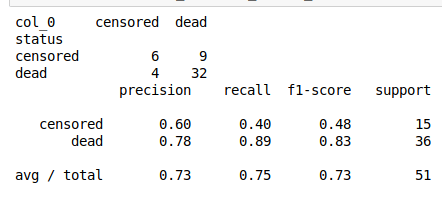Our overall accuracy is about 75%. How good this is depends in context. We are really good at predicting people are dead but have much more trouble with predicting if people are censored.

Conclusion

This post provided an example of using random forest in python. Through the use of a forest of trees, it is possible to get much more accurate results when a comparison is made to a single decision tree. This is one of many reasons for the use of random forest in machine learning.# Data Exploration Case Study: Credit Default

Exploratory data analysis is the main task of a Data Scientist with as much as 60% of their time being devoted to this task. As such, the majority of their time is spent on something that is rather boring compared to building models.This post will provide a simple example of how to analyze a dataset from the website called Kaggle. This dataset is looking at how is likely to default on their credit. The following steps will be conducted in this analysis.

1. Load the libraries and dataset
2. Deal with missing data
3. Some descriptive stats
4. Normality check
5. Model development

This is not an exhaustive analysis but rather a simple one for demonstration purposes. The dataset is available here

Load Libraries and Data

Here are some packages we will need

```import pandas as pd
import matplotlib.pyplot as plt
import seaborn as sns
from scipy.stats import norm
from sklearn import tree
from scipy import stats
from sklearn import metrics```

You can load the data with the code below

`df_train=pd.read_csv('/application_train.csv')`

You can examine what variables are available with the code below. This is not displayed here because it is rather long

```df_train.columns

Missing Data

I prefer to deal with missing data first because missing values can cause errors throughout the analysis if they are not dealt with immediately. The code below calculates the percentage of missing data in each column.

```total=df_train.isnull().sum().sort_values(ascending=False)
percent=(df_train.isnull().sum()/df_train.isnull().count()).sort_values(ascending=False)
missing_data=pd.concat([total,percent],axis=1,keys=['Total','Percent'])

Total   Percent
COMMONAREA_MEDI           214865  0.698723
COMMONAREA_AVG            214865  0.698723
COMMONAREA_MODE           214865  0.698723
NONLIVINGAPARTMENTS_MODE  213514  0.694330
NONLIVINGAPARTMENTS_MEDI  213514  0.694330```

Only the first five values are printed. You can see that some variables have a large amount of missing data. As such, they are probably worthless for inclusion in additional analysis. The code below removes all variables with any missing data.

```pct_null = df_train.isnull().sum() / len(df_train)
missing_features = pct_null[pct_null > 0.0].index
df_train.drop(missing_features, axis=1, inplace=True)```

You can use the .head() function if you want to see how  many variables are left.

Data Description & Visualization

For demonstration purposes, we will print descriptive stats and make visualizations of a few of the variables that are remaining.

```round(df_train['AMT_CREDIT'].describe())
Out:
count     307511.0
mean      599026.0
std       402491.0
min        45000.0
25%       270000.0
50%       513531.0
75%       808650.0
max      4050000.0

sns.distplot(df_train['AMT_CREDIT']```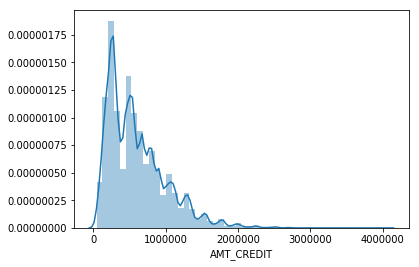```round(df_train['AMT_INCOME_TOTAL'].describe())
Out:
count       307511.0
mean        168798.0
std         237123.0
min          25650.0
25%         112500.0
50%         147150.0
75%         202500.0
max      117000000.0
sns.distplot(df_train['AMT_INCOME_TOTAL']```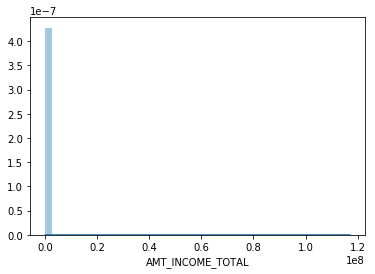I think you are getting the point. You can also look at categorical variables using the groupby() function.

We also need to address categorical variables in terms of creating dummy variables. This is so that we can develop a model in the future. Below is the code for dealing with all the categorical  variables and converting them to dummy variable’s

```df_train.groupby('NAME_CONTRACT_TYPE').count()
dummy=pd.get_dummies(df_train['NAME_CONTRACT_TYPE'])
df_train=pd.concat([df_train,dummy],axis=1)
df_train=df_train.drop(['NAME_CONTRACT_TYPE'],axis=1)

df_train.groupby('CODE_GENDER').count()
dummy=pd.get_dummies(df_train['CODE_GENDER'])
df_train=pd.concat([df_train,dummy],axis=1)
df_train=df_train.drop(['CODE_GENDER'],axis=1)

df_train.groupby('FLAG_OWN_CAR').count()
dummy=pd.get_dummies(df_train['FLAG_OWN_CAR'])
df_train=pd.concat([df_train,dummy],axis=1)
df_train=df_train.drop(['FLAG_OWN_CAR'],axis=1)

df_train.groupby('FLAG_OWN_REALTY').count()
dummy=pd.get_dummies(df_train['FLAG_OWN_REALTY'])
df_train=pd.concat([df_train,dummy],axis=1)
df_train=df_train.drop(['FLAG_OWN_REALTY'],axis=1)

df_train.groupby('NAME_INCOME_TYPE').count()
dummy=pd.get_dummies(df_train['NAME_INCOME_TYPE'])
df_train=pd.concat([df_train,dummy],axis=1)
df_train=df_train.drop(['NAME_INCOME_TYPE'],axis=1)

df_train.groupby('NAME_EDUCATION_TYPE').count()
dummy=pd.get_dummies(df_train['NAME_EDUCATION_TYPE'])
df_train=pd.concat([df_train,dummy],axis=1)
df_train=df_train.drop(['NAME_EDUCATION_TYPE'],axis=1)

df_train.groupby('NAME_FAMILY_STATUS').count()
dummy=pd.get_dummies(df_train['NAME_FAMILY_STATUS'])
df_train=pd.concat([df_train,dummy],axis=1)
df_train=df_train.drop(['NAME_FAMILY_STATUS'],axis=1)

df_train.groupby('NAME_HOUSING_TYPE').count()
dummy=pd.get_dummies(df_train['NAME_HOUSING_TYPE'])
df_train=pd.concat([df_train,dummy],axis=1)
df_train=df_train.drop(['NAME_HOUSING_TYPE'],axis=1)

df_train.groupby('ORGANIZATION_TYPE').count()
dummy=pd.get_dummies(df_train['ORGANIZATION_TYPE'])
df_train=pd.concat([df_train,dummy],axis=1)
df_train=df_train.drop(['ORGANIZATION_TYPE'],axis=1)```

You have to be careful with this because now you have many variables that are not necessary. For every categorical variable you must remove at least one category in order for the model to work properly.  Below we did this manually.

`df_train=df_train.drop(['Revolving loans','F','XNA','N','Y','SK_ID_CURR,''Student','Emergency','Lower secondary','Civil marriage','Municipal apartment'],axis=1)`

Below are some boxplots with the target variable and other variables in the dataset.

```f,ax=plt.subplots(figsize=(8,6))
fig=sns.boxplot(x=df_train['TARGET'],y=df_train['AMT_INCOME_TOTAL'])```There is a clear outlier there. Below is another boxplot with a different variable

```f,ax=plt.subplots(figsize=(8,6))
fig=sns.boxplot(x=df_train['TARGET'],y=df_train['CNT_CHILDREN'])```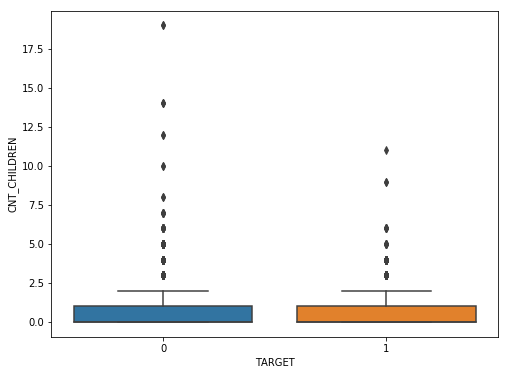It appears several people have more than 10 children. This is probably a typo.

Below is a correlation matrix using a heatmap technique

```corrmat=df_train.corr()
f,ax=plt.subplots(figsize=(12,9))
sns.heatmap(corrmat,vmax=.8,square=True)```The heatmap is nice but it is hard to really appreciate what is happening. The code below will sort the correlations from least to strongest, so we can remove high correlations.

```c = df_train.corr().abs()

s = c.unstack()
so = s.sort_values(kind="quicksort")

FLAG_DOCUMENT_12 FLAG_MOBIL 0.000005
FLAG_MOBIL FLAG_DOCUMENT_12 0.000005
Unknown FLAG_MOBIL 0.000005
FLAG_MOBIL Unknown 0.000005
Cash loans FLAG_DOCUMENT_14 0.000005```

The list is to long to show here but the following variables were removed for having a high correlation with other variables.

`df_train=df_train.drop(['WEEKDAY_APPR_PROCESS_START','FLAG_EMP_PHONE','REG_CITY_NOT_WORK_CITY','REGION_RATING_CLIENT','REG_REGION_NOT_WORK_REGION'],axis=1)`

Below we check a few variables for homoscedasticity, linearity, and normality  using plots and histograms

```sns.distplot(df_train['AMT_INCOME_TOTAL'],fit=norm)
fig=plt.figure()
res=stats.probplot(df_train['AMT_INCOME_TOTAL'],plot=plt)```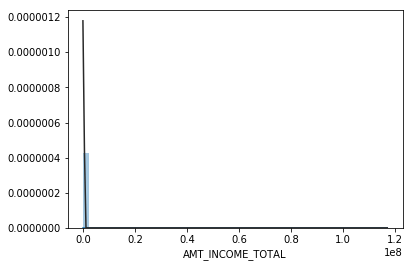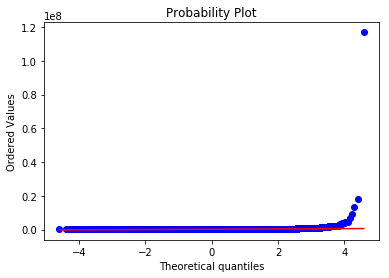This is not normal

```sns.distplot(df_train['AMT_CREDIT'],fit=norm)
fig=plt.figure()
res=stats.probplot(df_train['AMT_CREDIT'],plot=plt)```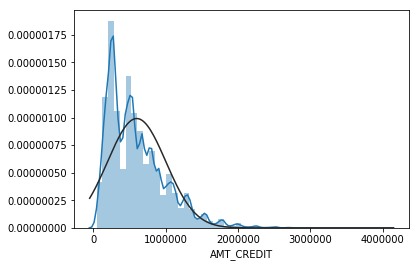This is not normal either. We could do transformations, or we can make a non-linear model instead.

Model Development

Now comes the easy part. We will make a decision tree using only some variables to predict the target. In the code below we make are X and y dataset.

```X=df_train[['Cash loans','DAYS_EMPLOYED','AMT_CREDIT','AMT_INCOME_TOTAL','CNT_CHILDREN','REGION_POPULATION_RELATIVE']]
y=df_train['TARGET']```

The code below fits are model and makes the predictions

```clf=tree.DecisionTreeClassifier(min_samples_split=20)
clf=clf.fit(X,y)
y_pred=clf.predict(X)```

Below is the confusion matrix followed by the accuracy

```print (pd.crosstab(y_pred,df_train['TARGET']))
TARGET       0      1
row_0
0       280873  18493
1         1813   6332
accuracy_score(y_pred,df_train['TARGET'])
Out: 0.933966589813047```

Lastly, we can look at the precision, recall, and f1 score

```print(metrics.classification_report(y_pred,df_train['TARGET']))
precision    recall  f1-score   support

0       0.99      0.94      0.97    299366
1       0.26      0.78      0.38      8145

micro avg       0.93      0.93      0.93    307511
macro avg       0.62      0.86      0.67    307511
weighted avg       0.97      0.93      0.95    307511```

This model looks rather good in terms of accuracy of the training set. It actually impressive that we could use so few variables from such a large dataset and achieve such a high degree of accuracy.

Conclusion

Data exploration and analysis is the primary task of a data scientist.  This post was just an example of how this can be approached. Of course, there are many other creative ways to do this but the simplistic nature of this analysis yielded strong results# RANSAC Regression in Python

RANSAC is an acronym for Random Sample Consensus. What this algorithm does is fit a regression model on a subset of data that the algorithm judges as inliers while removing outliers. This naturally improves the fit of the model due to the removal of some data points.The process that is used to determine inliers and outliers is described below.

1. The algorithm randomly selects a random amount of samples to be inliers in the model.
2. All data is used to fit the model and samples that fall with a certain tolerance are relabeled as inliers.
3. Model is refitted with the new inliers
4. Error of the fitted model vs the inliers is calculated
5. Terminate or go back to step 1 if a certain criterion of iterations or performance is not met.

In this post, we will use the tips data from the pydataset module. Our goal will be to predict the tip amount using two different models.

1. Model 1 will use simple regression and will include total bill as the independent variable and tips as the dependent variable
2. Model 2 will use multiple regression and  includes several independent variables and tips as the dependent variable

The process we will use to complete this example is as follows

1. Data preparation
2. Simple Regression Model fit
3. Simple regression visualization
4. Multiple regression model fit
5. Multiple regression visualization

Below are the packages we will need for this example

```import pandas as pd
from pydataset import data
from sklearn.linear_model import RANSACRegressor
from sklearn.linear_model import LinearRegression
import numpy as np
import matplotlib.pyplot as plt
from sklearn.metrics import mean_absolute_error
from sklearn.metrics import r2_score```

Data Preparation

For the data preparation, we need to do the following

1. Load the data
2. Create X and y dataframes
3. Convert several categorical variables to dummy variables
4. Drop the original categorical variables from the X dataframe

Below is the code for these steps

```df=data('tips')
X,y=df[['total_bill','sex','size','smoker','time']],df['tip']
male=pd.get_dummies(X['sex'])
X['male']=male['Male']
smoker=pd.get_dummies(X['smoker'])
X['smoker']=smoker['Yes']
dinner=pd.get_dummies(X['time'])
X['dinner']=dinner['Dinner']
X=X.drop(['sex','time'],1)```

Most of this is self-explanatory, we first load the tips dataset and divide the independent and dependent variables into an X and y dataframe respectively. Next, we converted the sex, smoker, and dinner variables into dummy variables, and then we dropped the original categorical variables.

We can now move to fitting the first model that uses simple regression.

Simple Regression Model

For our model, we want to use total bill to predict tip amount. All this is done in the following steps.

1. Instantiate an instance of the RANSACRegressor. We the call LinearRegression function, and we also set the residual_threshold to 2 indicate how far an example has to be away from  2 units away from the line.
2. Next we fit the model
3. We predict the values
4. We calculate the r square  the mean absolute error

Below is the code for all of this.

```ransacReg1= RANSACRegressor(LinearRegression(),residual_threshold=2,random_state=0)
ransacReg1.fit(X[['total_bill']],y)
prediction1=ransacReg1.predict(X[['total_bill']])```
```r2_score(y,prediction1)
Out: 0.4381748268686979

mean_absolute_error(y,prediction1)
Out: 0.7552429811944833```

The r-square is 44% while the MAE is 0.75.  These values are most comparative and will be looked at again when we create the multiple regression model.

The next step is to make the visualization. The code below will create a plot that shows the X and y variables and the regression. It also identifies which samples are inliers and outliers. Te coding will not be explained because of the complexity of it.

```inlier=ransacReg1.inlier_mask_
outlier=np.logical_not(inlier)
line_X=np.arange(3,51,2)
line_y=ransacReg1.predict(line_X[:,np.newaxis])
plt.scatter(X[['total_bill']][inlier],y[inlier],c='lightblue',marker='o',label='Inliers')
plt.scatter(X[['total_bill']][outlier],y[outlier],c='green',marker='s',label='Outliers')
plt.plot(line_X,line_y,color='black')
plt.xlabel('Total Bill')
plt.ylabel('Tip')
plt.legend(loc='upper left')```Plot is self-explanatory as a handful of samples were considered outliers. We will now move to creating our multiple regression model.

Multiple Regression Model Development

The steps for making the model are mostly the same. The real difference takes place in make the plot which we will discuss in a moment. Below is the code for  developing the model.

```ransacReg2= RANSACRegressor(LinearRegression(),residual_threshold=2,random_state=0)
ransacReg2.fit(X,y)
prediction2=ransacReg2.predict(X)```
```r2_score(y,prediction2)
Out: 0.4298703800652126

mean_absolute_error(y,prediction2)
Out: 0.7649733201032204```

Things have actually gotten slightly worst in terms of r-square and MAE.

For the visualization, we cannot plot directly several variables t once. Therefore, we will compare the predicted values with the actual values. The better the correlated the better our prediction is. Below is the code for the visualization

```inlier=ransacReg2.inlier_mask_
outlier=np.logical_not(inlier)
line_X=np.arange(1,8,1)
line_y=(line_X[:,np.newaxis])
plt.scatter(prediction2[inlier],y[inlier],c='lightblue',marker='o',label='Inliers')
plt.scatter(prediction2[outlier],y[outlier],c='green',marker='s',label='Outliers')
plt.plot(line_X,line_y,color='black')
plt.xlabel('Predicted Tip')
plt.ylabel('Actual Tip')
plt.legend(loc='upper left')```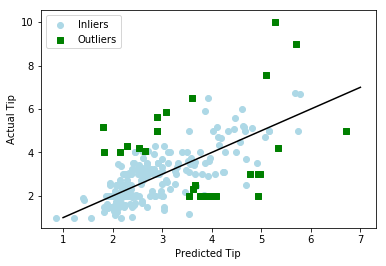The plots are mostly the same  as you cans see for yourself.

Conclusion

This post provided an example of how to use the RANSAC regressor algorithm. This algorithm will remove samples from the model based on a criterion you set. The biggest complaint about this algorithm is that it removes data from the model. Generally, we want to avoid losing data when developing models. In addition, the algorithm removes outliers objectively this is a problem because outlier removal is often subjective. Despite these flaws, RANSAC regression is another tool that can be use din machine learning.# Combining Algorithms for Classification with Python

Many approaches in machine learning involve making many models that combine their strength and weaknesses to make more accuracy classification. Generally, when this is done it is the same algorithm being used. For example, random forest is simply many decision trees being developed. Even when bagging or boosting is being used it is the same algorithm but with variances in sampling and the use of features.In addition to this common form of ensemble learning there is also a way to combine different algorithms to make predictions. For one way of doing this is through a technique called stacking in which the predictions of several models are passed to a higher model that uses the individual model predictions to make a final prediction. In this post we will look at how to do this using Python.

Assumptions

This blog usually tries to explain as much  as possible about what is happening. However, due to the complexity of this topic there are several assumptions about the reader’s background.

• Already familiar with python
• Can use various algorithms to make predictions (logistic regression, linear discriminant analysis, decision trees, K nearest neighbors)
• Familiar with cross-validation and hyperparameter tuning

We will be using the Mroz dataset in the pydataset module. Our goal is to use several of the independent variables to predict whether someone lives in the city or not.

The steps we will take in this post are as follows

1. Data preparation
2. Individual model development
3. Ensemble model development
4. Hyperparameter tuning of ensemble model
5. Ensemble model testing

Below is all of the libraries we will be using in this post

```import pandas as pd
from sklearn.model_selection import GridSearchCV
from sklearn.preprocessing import StandardScaler
from sklearn.preprocessing import LabelEncoder
from pydataset import data
from sklearn.model_selection import train_test_split
from sklearn.model_selection import cross_val_score
from sklearn.linear_model import LogisticRegression
from sklearn.tree import DecisionTreeClassifier
from sklearn.neighbors import KNeighborsClassifier
from mlxtend.classifier import EnsembleVoteClassifier
from sklearn.discriminant_analysis import LinearDiscriminantAnalysis as LDA
from sklearn.metrics import accuracy_score
from sklearn.metrics import classification_report```

Data Preparation

We need to perform the following steps for the data preparation

1. Load the data
2. Select the independent variables to be used in the analysis
3. Scale the independent variables
4. Convert the dependent variable from text to numbers
5. Split the data in train and test sets

Not all of the variables in the Mroz dataset were used. Some were left out because they were highly correlated with others. This analysis is not in this post but you can explore this on your own. The data was also scaled because many algorithms are sensitive to this so it is best practice to always scale the data. We will use the StandardScaler function for this. Lastly, the dpeendent variable currently consist of values of “yes” and “no” these need to be convert to numbers 1 and 0. We will use the LabelEncoder function for this. The code for all of this is below.

```df=data('Mroz')
X,y=df[['hoursw','child6','child618','educw','hearnw','hoursh','educh','wageh','educwm','educwf','experience']],df['city']
sc=StandardScaler()
X_scale=sc.fit_transform(X)
X=pd.DataFrame(X_scale, index=X.index, columns=X.columns)
le=LabelEncoder()
y=le.fit_transform(y)
X_train, X_test,y_train, y_test=train_test_split(X,y,test_size=.3,random_state=5)

```

We can now proceed to individul model development

Individual Model Development

Below are the steps for this part of the analysis

1. Instantiate an instance of each algorithm
2. Check accuracy of each model
3. Check roc curve of each model

We will create four different models, and they are logistic regression, decision tree, k nearest neighbor, and linear discriminant analysis. We will also set some initial values for the hyperparameters for each. Below is the code

```logclf=LogisticRegression(penalty='l2',C=0.001, random_state=0)
treeclf=DecisionTreeClassifier(max_depth=3,criterion='entropy',random_state=0)
knnclf=KNeighborsClassifier(n_neighbors=5,p=2,metric='minkowski')
LDAclf=LDA()```

We can now assess the accuracy and roc curve of each model. This will be done through using two separate for loops. The first will have the accuracy results and the second will have the roc curve results. The results will also use k-fold cross validation with the cross_val_score function. Below is the code with the results.

```clf_labels=['Logistic Regression','Decision Tree','KNN','LDAclf']
for clf, label in zip ([logclf,treeclf,knnclf,LDAclf],clf_labels):
scores=cross_val_score(estimator=clf,X=X_train,y=y_train,cv=10,scoring='accuracy')
print("accuracy: %0.2f (+/- %0.2f) [%s]" % (scores.mean(),scores.std(),label))

for clf, label in zip ([logclf,treeclf,knnclf,LDAclf],clf_labels):
scores=cross_val_score(estimator=clf,X=X_train,y=y_train,cv=10,scoring='roc_auc')
print("roc auc: %0.2f (+/- %0.2f) [%s]" % (scores.mean(),scores.std(),label))

accuracy: 0.69 (+/- 0.04) [Logistic Regression]
accuracy: 0.72 (+/- 0.06) [Decision Tree]
accuracy: 0.66 (+/- 0.06) [KNN]
accuracy: 0.70 (+/- 0.05) [LDAclf]
roc auc: 0.71 (+/- 0.08) [Logistic Regression]
roc auc: 0.70 (+/- 0.07) [Decision Tree]
roc auc: 0.62 (+/- 0.10) [KNN]
roc auc: 0.70 (+/- 0.08) [LDAclf]```

The results can speak for themselves. We have a general accuracy of around 70% but our roc auc is poor. Despite this we will now move to the ensemble model development.

Ensemble Model Development

The ensemble model requires the use of the EnsembleVoteClassifier function. Inside this function are the four models we made earlier. Other than this the rest of the code is the same as the previous step. We will assess the accuracy and the roc auc. Below is the code and the results

``` mv_clf= EnsembleVoteClassifier(clfs=[logclf,treeclf,knnclf,LDAclf],weights=[1.5,1,1,1])

for clf, label in zip ([logclf,treeclf,knnclf,LDAclf,mv_clf],labels):
scores=cross_val_score(estimator=clf,X=X_train,y=y_train,cv=10,scoring='accuracy')
print("accuracy: %0.2f (+/- %0.2f) [%s]" % (scores.mean(),scores.std(),label))

for clf, label in zip ([logclf,treeclf,knnclf,LDAclf,mv_clf],labels):
scores=cross_val_score(estimator=clf,X=X_train,y=y_train,cv=10,scoring='roc_auc')
print("roc auc: %0.2f (+/- %0.2f) [%s]" % (scores.mean(),scores.std(),label))

accuracy: 0.69 (+/- 0.04) [LR]
accuracy: 0.72 (+/- 0.06) [tree]
accuracy: 0.66 (+/- 0.06) [knn]
accuracy: 0.70 (+/- 0.05) [LDA]
accuracy: 0.70 (+/- 0.04) [combine]
roc auc: 0.71 (+/- 0.08) [LR]
roc auc: 0.70 (+/- 0.07) [tree]
roc auc: 0.62 (+/- 0.10) [knn]
roc auc: 0.70 (+/- 0.08) [LDA]
roc auc: 0.72 (+/- 0.09) [combine]```

You can see that the combine model as similar performance to the individual models. This means in this situation that the ensemble learning did not make much of a difference. However, we have not tuned are hyperparameters yet. This will be done in the next step.

Hyperparameter Tuning of Ensemble Model

We are going to tune the decision tree, logistic regression, and KNN model. There are many different hyperparameters we can tune. For demonstration purposes we are only tuning one hyperparameter per algorithm. Once we set the hyperparameters we will run the model and pull the best hyperparameters values based on the roc auc as the metric. Below is the code and the output.

```params={'decisiontreeclassifier__max_depth':[2,3,5],
'logisticregression__C':[0.001,0.1,1,10],
'kneighborsclassifier__n_neighbors':[5,7,9,11]}

grid=GridSearchCV(estimator=mv_clf,param_grid=params,cv=10,scoring='roc_auc')

grid.fit(X_train,y_train)

grid.best_params_
Out:
{'decisiontreeclassifier__max_depth': 3,
'kneighborsclassifier__n_neighbors': 9,
'logisticregression__C': 10}

grid.best_score_
Out: 0.7196051482279385```

The best values are as follows

• Decision tree max depth set to 3
• KNN number of neighbors set to 9
• logistic regression C set to 10

These values give us a roc auc of 0.72 which is still poor . We can now use these values when we test our final model.

Ensemble Model Testing

The following steps are performed in the analysis

1. Created new instances of the algorithms with the adjusted hyperparameters
2. Run the ensemble model
3. Predict with the test data
4. Check the results

Below is the first step

```logclf=LogisticRegression(penalty='l2',C=10, random_state=0)
treeclf=DecisionTreeClassifier(max_depth=3,criterion='entropy',random_state=0)
knnclf=KNeighborsClassifier(n_neighbors=9,p=2,metric='minkowski')
LDAclf=LDA()```

Below is step two

```mv_clf= EnsembleVoteClassifier(clfs=[logclf,treeclf,knnclf,LDAclf],weights=[1.5,1,1,1])
mv_clf.fit(X_train,y_train)```

Below are steps 3 and four

```y_pred=mv_clf.predict(X_test)
print(accuracy_score(y_test,y_pred))
print(pd.crosstab(y_test,y_pred))
print(classification_report(y_test,y_pred))

0.6902654867256637
col_0   0    1
row_0
0      29   58
1      12  127
precision    recall  f1-score   support

0       0.71      0.33      0.45        87
1       0.69      0.91      0.78       139

avg / total       0.69      0.69      0.66       226```

The accuracy is about 69%. One thing that is noticeable low is the  recall for people who do not live in the city. This probably one reason why the overall roc auc score is so low. The f1-score is also low for those who do not live in the city as well. The f1-score is just a combination of precision and recall. If we really want to improve performance we would probably start with improving the recall of the no’s.

Conclusion

This post provided an example of how you can  combine different algorithms to make predictions in Python. This is a powerful technique t to use. Off course, it is offset by the complexity of the analysis which makes it hard to explain exactly what the results mean if you were asked tot do so.# Gradient Boosting Regression in Python

In this  post, we will take a look at gradient boosting for regression. Gradient boosting simply makes sequential models that try to explain any examples that had not been explained by previously models. This approach makes gradient boosting superior to AdaBoost.Regression trees are mostly commonly teamed with boosting. There are some additional hyperparameters that need to be set  which includes the following

• number of estimators
• learning rate
• subsample
• max depth

We will deal with each of these when it is appropriate. Our goal in this post is to predict the amount of weight loss in cancer patients based on the independent variables. This is the process we will follow to achieve this.

1. Data preparation
2. Baseline decision tree model
3. Hyperparameter tuning
4. Gradient boosting model development

Below is some initial code

```from sklearn.ensemble import GradientBoostingRegressor
from sklearn import tree
from sklearn.model_selection import GridSearchCV
import numpy as np
from pydataset import data
import pandas as pd
from sklearn.model_selection import cross_val_score
from sklearn.model_selection import KFold```

Data Preparation

The data preparation is not that difficult in this situation. We simply need to load the dataset in an object and remove any missing values. Then we separate the independent and dependent variables into separate datasets. The code is below.

```df=data('cancer').dropna()
X=df[['time','sex','ph.karno','pat.karno','status','meal.cal']]
y=df['wt.loss']```

We can now move to creating our baseline model.

Baseline Model

The purpose of the baseline model is to have something to compare our gradient boosting model to. Therefore, all we will do here is create  several regression trees. The difference between the regression trees will be the max depth. The max depth has to with the number of nodes python can make to try to purify the classification.  We will then decide which tree is best based on the mean squared error.

The first thing we need to do is set the arguments for the cross-validation. Cross validating the results helps to check the accuracy of the results. The rest of the code  requires the use of for loops and if statements that cannot be reexplained in this post. Below is the code with the output.

`crossvalidation=KFold(n_splits=10,shuffle=True,random_state=1)`
```for depth in range (1,10):
tree_regressor=tree.DecisionTreeRegressor(max_depth=depth,random_state=1)
if tree_regressor.fit(X,y).tree_.max_depth<depth:
break
score=np.mean(cross_val_score(tree_regressor,X,y,scoring='neg_mean_squared_error', cv=crossvalidation,n_jobs=1))
print(depth, score)```
``` 1 -193.55304528235052
2 -176.27520747356175
3 -209.2846723461564
4 -218.80238479654003
5 -222.4393459885871
6 -249.95330609042858
7 -286.76842138165705
8 -294.0290706405905
9 -287.39016236497804```

You can see that a max depth of 2 had the lowest amount of error. Therefore, our baseline model has a mean squared error of 176. We need to improve on this in order to say that our gradient boosting model is superior.

However, before we create our gradient boosting model. we need to tune the hyperparameters of the algorithm.

Hyperparameter Tuning

Hyperparameter tuning has to with setting the value of parameters that the algorithm cannot learn on its own. As such, these are constants that you set as the researcher. The problem is that you are not any better at knowing where to set these values than the computer. Therefore, the process that is commonly used is to have the algorithm use several combinations  of values until it finds the values that are best for the model/. Having said this, there are several hyperparameters we need to tune, and they are as follows.

• number of estimators
• learning rate
• subsample
• max depth

The number of estimators is show many trees to create. The more trees the more likely to overfit. The learning rate is the weight that each tree has on the final prediction. Subsample is the proportion of the sample to use. Max depth was explained previously.

What we will do now is make an instance of the GradientBoostingRegressor. Next, we will create our grid with the various values for the hyperparameters. We will then take this grid and place it inside GridSearchCV function so that we can prepare to run our model. There are some arguments that need to be set inside the GridSearchCV function such as estimator, grid, cv, etc. Below is the code.

```GBR=GradientBoostingRegressor()
search_grid={'n_estimators':[500,1000,2000],'learning_rate':[.001,0.01,.1],'max_depth':[1,2,4],'subsample':[.5,.75,1],'random_state':}
search=GridSearchCV(estimator=GBR,param_grid=search_grid,scoring='neg_mean_squared_error',n_jobs=1,cv=crossvalidation)```

We can now run the code and determine the best combination of hyperparameters and how well the model did base on the means squared error metric. Below is the code and the output.

```search.fit(X,y)
search.best_params_
Out:
{'learning_rate': 0.01,
'max_depth': 1,
'n_estimators': 500,
'random_state': 1,
'subsample': 0.5}

search.best_score_
Out: -160.51398257591643```

The hyperparameter results speak for themselves. With this tuning we can see that the mean squared error is lower than with the baseline model. We can now move to the final step of taking these hyperparameter settings and see how they do on the dataset. The results should be almost the same.

Gradient Boosting Model Development

Below is the code and the output for the tuned gradient boosting model

```GBR2=GradientBoostingRegressor(n_estimators=500,learning_rate=0.01,subsample=.5,max_depth=1,random_state=1)
score=np.mean(cross_val_score(GBR2,X,y,scoring='neg_mean_squared_error',cv=crossvalidation,n_jobs=1))
score
Out: -160.77842893572068```

These results were to be expected. The gradient boosting model has a better performance than the baseline regression tree model.

Conclusion

In this post, we looked at how to  use gradient boosting to improve a regression tree. By creating multiple models. Gradient boosting will almost certainly have a better performance than other type of algorithms that rely on only one model.# Gradient Boosting Classification in Python

Gradient Boosting is an alternative form of boosting to AdaBoost. Many consider gradient boosting to be a better performer than adaboost. Some differences between the two algorithms is that gradient boosting uses optimization for weight the estimators. Like adaboost, gradient boosting can be used for most algorithms but is commonly associated with decision trees.

In addition, gradient boosting requires several additional hyperparameters such as max depth and subsample. Max depth has to do with the number of nodes in a tree. The higher the number the purer the classification become. The downside to this is the risk of overfitting.

Subsampling has to do with the proportion of the sample that is used for each estimator. This can range from a decimal value up until the whole number 1. If the value is set to 1 it becomes stochastic gradient boosting.

This post is focused on classification. To do this, we will use the cancer dataset from the pydataset library. Our goal will be to predict the status of patients (alive or dead) using the available independent variables. The steps we will use are as follows.

1. Data preparation
2. Baseline decision tree model
3. Hyperparameter tuning
4. Gradient boosting model development

Below is some initial code.

`from sklearn.ensemble import GradientBoostingClassifier from sklearn import tree from sklearn.model_selection import GridSearchCV import numpy as np from pydataset import data import pandas as pd from sklearn.model_selection import cross_val_score from sklearn.model_selection import KFold`

Data Preparation

The data preparation is simple in this situtation. All we need to do is load are dataset, dropping missing values, and create our X dataset and y dataset. All this happens in the code below.

`df=data('cancer').dropna() X=df[['time','sex','ph.karno','pat.karno','meal.cal','wt.loss']] y=df['status'] `

We will now develop our baseline decision tree model.

Baseline Model

The purpose of the baseline model is to have something to compare our gradient boosting model to. The strength of a model is always relative to some other model, so we need to make at least two, so we can say one is better than the other.

The criteria for better in this situation is accuracy. Therefore, we will make a decision tree model, but we will manipulate the max depth of the tree to create 9 different baseline models. The best accuracy model will be the baseline model.

To achieve this, we need to use a for loop to make python make several decision trees. We also need to set the parameters for the cross validation by calling KFold(). Once this is done, we print the results for the 9 trees. Below is the code and results.

`crossvalidation=KFold(n_splits=10,shuffle=True,random_state=1)for depth in range (1,10):     tree_classifier=tree.DecisionTreeClassifier(max_depth=depth,random_state=1)     if tree_classifier.fit(X,y).tree_.max_depth<depth:         break     score=np.mean(cross_val_score(tree_classifier,X,y,scoring='accuracy', cv=crossvalidation,n_jobs=1))     print(depth, score) 1 0.71875 2 0.6477941176470589 3 0.6768382352941177 4 0.6698529411764707 5 0.6584558823529412 6 0.6525735294117647 7 0.6283088235294118 8 0.6573529411764706 9 0.6577205882352941`

It appears that when the max depth is limited to 1 that we get the best accuracy at almost 72%. This will be our baseline for comparison. We will now tune the parameters for the gradient boosting algorithm

Hyperparameter Tuning

There are several hyperparameters we need to tune. The ones we will tune are as follows

• number of estimators
• learning rate
• subsample
• max depth

First, we will create an instance of the gradient boosting classifier. Second, we will create our grid for the search. It is inside this grid that we set several values for each hyperparameter. Then we call GridSearchCV and place the instance of the gradient boosting classifier, the grid, the cross validation values from mad earlier, and n_jobs all together in one place. Below is the code for this.

`GBC=GradientBoostingClassifier() search_grid={'n_estimators':[500,1000,2000],'learning_rate':[.001,0.01,.1],'max_depth':[1,3,5],'subsample':[.5,.75,1],'random_state':}search=GridSearchCV(estimator=GBC,param_grid=search_grid,scoring='accuracy',n_jobs=1,cv=crossvalidation)`

You can now run your model by calling .fit(). Keep in mind that there are several hyperparameters. This means that it might take some time to run the calculations. It is common to find values for max depth, subsample, and number of estimators first. Then as second run through is done to find the learning rate. In our example, we are doing everything at once which is why it takes longer. Below is the code with the out for best parameters and best score.

`search.fit(X,y)search.best_params_ Out:  {'learning_rate': 0.01,  'max_depth': 5,  'n_estimators': 2000,  'random_state': 1,  'subsample': 0.75} search.best_score_ Out: 0.7425149700598802`

You can see what the best hyperparameters are for yourself. In addition, we see that when these parameters were set we got an accuracy of 74%. This is superior to our baseline model. We will now see if we can replicate these numbers when we use them for our Gradient Boosting model.

Below is the code and results for the model with the predetermined hyperparameter values.

`ada2=GradientBoostingClassifier(n_estimators=2000,learning_rate=0.01,subsample=.75,max_depth=5,random_state=1) score=np.mean(cross_val_score(ada2,X,y,scoring='accuracy',cv=crossvalidation,n_jobs=1)) score Out: 0.742279411764706`

You can see that the results are similar. This is just additional information that the gradient boosting model does outperform the baseline decision tree model.

Conclusion

This post provided an example of what gradient boosting classification can do for a model. With its distinct characteristics gradient boosting is generally a better performing boosting algorithm in comparison to AdaBoost.# AdaBoost Regression with Python

This post will share how to use the adaBoost algorithm for regression in Python. What boosting does is that it makes multiple models in a sequential manner. Each newer model tries to successful predict what older models struggled with. For regression, the average of the models are used for the predictions.  It is often most common to use boosting with decision trees but this approach can be used with any machine learning algorithm that deals with supervised learning.

Boosting is associated with ensemble learning because several models are created that are averaged together. An assumption of boosting, is that combining several weak models can make one really strong and accurate model.

For our purposes, we will be using adaboost classification to improve the performance of a decision tree in python. We will use the cancer dataset from the pydataset library. Our goal will be to predict the weight loss of a patient based on several independent variables. The steps of this process are as follows.

1. Data preparation
2. Regression decision tree baseline model
3. Hyperparameter tuning of Adaboost regression model
4. AdaBoost regression model development

Below is some initial code

`from sklearn.ensemble import AdaBoostRegressor from sklearn import tree from sklearn.model_selection import GridSearchCV import numpy as np from pydataset import data import pandas as pd from sklearn.model_selection import cross_val_score from sklearn.model_selection import train_test_split from sklearn.model_selection import KFold from sklearn.metrics import mean_squared_error`

Data Preparation

There is little data preparation for this example. All we need to do is load the data and create the X and y datasets. Below is the code.

`df=data('cancer').dropna() X=df[['time','sex','ph.karno','pat.karno','status','meal.cal']] y=df['wt.loss']`

We will now proceed to creating the baseline regression decision tree model.

Baseline Regression Tree Model

The purpose of the baseline model is to compare it to the performance of our model that utilizes adaBoost. To make this model we need to Initiate a K-fold cross-validation. This will help in stabilizing the results. Next, we will create a for loop to create several trees that vary based on their depth. By depth, it is meant how far the tree can go to purify the classification. More depth often leads to a higher likelihood of overfitting.

Finally, we will then print the results for each tree. The criteria used for judgment is the mean squared error. Below is the code and results

`crossvalidation=KFold(n_splits=10,shuffle=True,random_state=1)for depth in range (1,10):     tree_regressor=tree.DecisionTreeRegressor(max_depth=depth,random_state=1)     if tree_regressor.fit(X,y).tree_.max_depth<depth:         break     score=np.mean(cross_val_score(tree_regressor,X,y,scoring='neg_mean_squared_error', cv=crossvalidation,n_jobs=1))     print(depth, score) 1 -193.55304528235052 2 -176.27520747356175 3 -209.2846723461564 4 -218.80238479654003 5 -222.4393459885871 6 -249.95330609042858 7 -286.76842138165705 8 -294.0290706405905 9 -287.39016236497804`

Looks like a tree with a depth of 2 had the lowest amount of error. We can now move to tuning the hyperparameters for the adaBoost algorithm.

Hyperparameter Tuning

For hyperparameter tuning we need to start by initiating our AdaBoostRegresor() class. Then we need to create our grid. The grid will address two hyperparameters which are the number of estimators and the learning rate. The number of estimators tells Python how many models to make and the learning indicates how each tree contributes to the overall results. There is one more parameter which is random_state, but this is just for setting the seed and never changes.

After making the grid, we need to use the GridSearchCV function to finish this process. Inside this function, you have to set the estimator, which is adaBoostRegressor, the parameter grid which we just made, the cross-validation which we made when we created the baseline model, and the n_jobs, which allocates resources for the calculation. Below is the code.

`ada=AdaBoostRegressor()search_grid={'n_estimators':[500,1000,2000],'learning_rate':[.001,0.01,.1],'random_state':}search=GridSearchCV(estimator=ada,param_grid=search_grid,scoring='neg_mean_squared_error',n_jobs=1,cv=crossvalidation) `

Next, we can run the model with the desired grid in place. Below is the code for fitting the mode as well as the best parameters and the score to expect when using the best parameters.

`search.fit(X,y)search.best_params_ Out: {'learning_rate': 0.01, 'n_estimators': 500, 'random_state': 1} search.best_score_ Out: -164.93176650920856`

The best mix of hyperparameters is a learning rate of 0.01 and 500 estimators. This mix led to a mean error score of 164, which is a little lower than our single decision tree of 176. We will see how this works when we run our model with refined hyperparameters.

Below is our model, but this time with the refined hyperparameters.

`ada2=AdaBoostRegressor(n_estimators=500,learning_rate=0.001,random_state=1)score=np.mean(cross_val_score(ada2,X,y,scoring='neg_mean_squared_error',cv=crossvalidation,n_jobs=1)) score Out: -174.52604137201791`

You can see the score is not as good but it is within reason.

Conclusion

In this post, we explored how to use the AdaBoost algorithm for regression. Employing this algorithm can help to strengthen a model in many ways at times.# AdaBoost Classification in Python

Boosting is a technique in machine learning in which multiple models are developed sequentially. Each new model tries to successful predict what prior models were unable to do. The average for regression and majority vote for classification are used. For classification, boosting is commonly associated with decision trees. However, boosting can be used with any machine learning algorithm in the supervised learning context.

Since several models are being developed with aggregation, boosting is associated with ensemble learning. Ensemble is just a way of developing more than one model for machine-learning purposes. With boosting, the assumption is that the combination of several weak models can make one really strong and accurate model.

For our purposes, we will be using adaboost classification to improve the performance of a decision tree in python. We will use the cancer dataset from the pydataset library. Our goal will be to predict the status of a patient based on several independent variables. The steps of this process are as follows.

1. Data preparation
2. Decision tree baseline model
3. Hyperparameter tuning of Adaboost model
4. AdaBoost model development

Below is some initial code

`from sklearn.ensemble import AdaBoostClassifierfrom sklearn import treefrom sklearn.model_selection import GridSearchCVimport numpy as npfrom pydataset import dataimport pandas as pdfrom sklearn.model_selection import cross_val_scorefrom sklearn.model_selection import KFold`

Data Preparation

Data preparation is minimal in this situation. We will load are data and at the same time drop any NA using the .dropna() function. In addition, we will place the independent variables in dataframe called X and the dependent variable in a dataset called y. Below is the code.

`df=data('cancer').dropna()X=df[['time','sex','ph.karno','pat.karno','meal.cal','wt.loss']]y=df['status']`

Decision Tree Baseline Model

We will make a decision tree just for the purposes of comparison. First, we will set the parameters for the cross-validation. Then we will use a for loop to run several different decision trees. The difference in the decision trees will be their depth. The depth is how far the tree can go in order to purify the classification. The more depth the more likely your decision tree is to overfit the data. The last thing we will do is print the results. Below is the code with the output

`crossvalidation=KFold(n_splits=10,shuffle=True,random_state=1)for depth in range (1,10):    tree_classifier=tree.DecisionTreeClassifier(max_depth=depth,random_state=1)    if tree_classifier.fit(X,y).tree_.max_depth<depth:        break    score=np.mean(cross_val_score(tree_classifier,X,y,scoring='accuracy', cv=crossvalidation,n_jobs=1))    print(depth, score)1 0.718752 0.64779411764705893 0.67683823529411774 0.66985294117647075 0.65845588235294126 0.65257352941176477 0.62830882352941188 0.65735294117647069 0.6577205882352941`

You can see that the most accurate decision tree had a depth of 1. After that there was a general decline in accuracy.

We now can determine if the adaBoost model is better based on whether the accuracy is above 72%. Before we develop the  AdaBoost model, we need to tune several hyperparameters in order to develop the most accurate model possible.

Hyperparameter Tuning AdaBoost Model

In order to tune the hyperparameters there are several things that we need to do. First we need to initiate  our AdaBoostClassifier with some basic settings. Then We need to create our search grid with the hyperparameters. There are two hyperparameters that we will set and they are number of estimators (n_estimators) and the learning rate.

Number of estimators has to do with how many trees are developed. The learning rate indicates how each tree contributes to the overall results. We have to place in the grid several values for each of these. Once we set the arguments for the AdaBoostClassifier and the search grid we combine all this information into an object called search. This object uses the GridSearchCV function and includes additional arguments for scoring, n_jobs, and for cross-validation. Below is the code for all of this

`ada=AdaBoostClassifier()search_grid={'n_estimators':[500,1000,2000],'learning_rate':[.001,0.01,.1]}search=GridSearchCV(estimator=ada,param_grid=search_grid,scoring='accuracy',n_jobs=1,cv=crossvalidation)`

We can now run the model of hyperparameter tuning and see the results. The code is below.

`search.fit(X,y)search.best_params_Out: {'learning_rate': 0.01, 'n_estimators': 1000}search.best_score_Out: 0.7425149700598802`

We can see that if the learning rate is set to 0.01 and the number of estimators to 1000 We can expect an accuracy of 74%. This is superior to our baseline model.

We can now rune our AdaBoost Classifier based on the recommended hyperparameters. Below is the code.

```ada=AdaBoostClassifier(n_estimators=1000,learning_rate=0.01)
score
Out: 0.7415441176470589```

We knew we would get around 74% and that is what we got. It’s only a 3% improvement but depending on the context that can be a substantial difference.

Conclusion

In this post, we look at how to use boosting for classification. In particular, we used the AdaBoost algorithm. Boosting in general uses many models to determine the most accurate classification in a sequential manner. Doing this will often lead to an improvement in the prediction of a model.# Recommendation Engine with Python

Recommendation engines make future suggestion to a person based on their prior behavior. There are several ways to develop recommendation engines but for purposes, we will be looking at the development of a user-based collaborative filter. This type of filter takes the ratings of others to suggest future items to another user based on the other user’s ratings.

Making a recommendation engine in Python actually does not take much code and is somewhat easy consider what can be done through coding. We will make a movie recommendation engine using data from movielens.

Inside the zip file are several files we will use. We will use each in a few moments. Below is the initial code to get started

``````import pandas as pd
from scipy.sparse import csr_matrix
from sklearn.decomposition import TruncatedSVD
import numpy as np``````

We will now make 4 dataframes. Dataframes 1-3 will be the user, rating, and movie title data. The last dataframe will be a merger of the first 3. The code is below with a printout of the final result.

``````user = pd.read_table('/home/darrin/Documents/python/new/ml-1m/users.dat', sep='::', header=None, names=['user_id', 'gender', 'age', 'occupation', 'zip'],engine='python')
rating = pd.read_table('/home/darrin/Documents/python/new/ml-1m/ratings.dat', sep='::', header=None, names=['user_id', 'movie_id', 'rating', 'timestamp'],engine='python')
movie = pd.read_table('/home/darrin/Documents/python/new/ml-1m/movies.dat', sep='::', header=None, names=['movie_id', 'title', 'genres'],engine='python')
MovieAll = pd.merge(pd.merge(rating, user), movie)``````

We now need to create a matrix using the .pivot_table function. This matrix will include ratings and user_id from our “MovieAll” dataframe. We will then move this information into a dataframe called “movie_index”. This index will help us keep track of what movie each column represents. The code is below.

``rating_mtx_df = MovieAll.pivot_table(values='rating', index='user_id', columns='title', fill_value=0)``

There are many variables in our matrix. This makes the computational time long and expensive. To reduce this we will reduce the dimensions using the TruncatedSVD function. We will reduce the matrix to 20 components. We also need to transform the data because we want the Vh matrix and no tthe U matrix. All this is hand in the code below.

``````recomm = TruncatedSVD(n_components=20, random_state=10)
R = recomm.fit_transform(rating_mtx_df.values.T)``````

What we saved our modified dataset as “R”. If we were to print this it would show that each row has two columns with various numbers in it that cannot be interpreted by us.  Instead, we will move to the actual recommendation part of this post.

To get a recommendation you have to tell Python the movie that you watch first. Python will then compare this movie with other movies that have a similiar rating and genera in the training dataset and then provide recommendation based on which movies have the highest correlation to the movie that was watched.

We are going to tell Python that we watched “One Flew Over the Cuckoo’s Nest” and see what movies it recommends.

First, we need to pull the information for just “One Flew Over the Cuckoo’s Nest”  and place this in a matrix. Then we need to calculate the correlations of all our movies using the modified dataset we named “R”. These two steps are completed below.

``````cuckoo_idx = list(movie_index).index("One Flew Over the Cuckoo's Nest (1975)")
correlation_matrix = np.corrcoef(R)``````

Now we can determine which movies have the highest correlation with our movie. However, to determine this, we must gvive Python a range of acceptable correlations. For our purposes we will set this between 0.93 and 1.0. The code is below with the recommendations.

``````P = correlation_matrix[cuckoo_idx]
print (list(movie_index[(P > 0.93) & (P < 1.0)]))
['Graduate, The (1967)', 'Taxi Driver (1976)']``````

You can see that the engine recommended two movies which are “The Graduate” and “Taxi Driver”. We could increase the number of recommendations by lower the correlation requirement if we desired.

Conclusion

Recommendation engines are a great tool for generating sales automatically for customers. Understanding the basics of how to do this a practical application of machine learning# Elastic Net Regression in Python

Elastic net regression combines the power of ridge and lasso regression into one algorithm. What this means is that with elastic net the algorithm can remove weak variables altogether as with lasso or to reduce them to close to zero as with ridge. All of these algorithms are examples of regularized regression.

This post will provide an example of elastic net regression in Python. Below are the steps of the analysis.

1. Data preparation
2. Baseline model development
3. Elastic net model development

To accomplish this, we will use the Fair dataset from the pydataset library. Our goal will be to predict marriage satisfaction based on the other independent variables. Below is some initial code to begin the analysis.

`from pydataset import dataimport numpy as npimport pandas as pdpd.set_option('display.max_rows', 5000)pd.set_option('display.max_columns', 5000)pd.set_option('display.width', 10000)from sklearn.model_selection import GridSearchCVfrom sklearn.linear_model import ElasticNetfrom sklearn.linear_model import LinearRegressionfrom sklearn.metrics import mean_squared_error`

Data Preparation

We will now load our data. The only preparation that we need to do is convert the factor variables to dummy variables. Then we will make our  and y datasets. Below is the code.

`df=pd.DataFrame(data('Fair'))df.loc[df.sex== 'male', 'sex'] = 0df.loc[df.sex== 'female','sex'] = 1df['sex'] = df['sex'].astype(int)df.loc[df.child== 'no', 'child'] = 0df.loc[df.child== 'yes','child'] = 1df['child'] = df['child'].astype(int)X=df[['religious','age','sex','ym','education','occupation','nbaffairs']]y=df['rate']`

We can now proceed to creating the baseline model

Baseline Model

This model is a basic regression model for the purpose of comparison. We will instantiate our regression model, use the fit command and finally calculate the mean squared error of the data. The code is below.

`regression=LinearRegression()regression.fit(X,y)first_model=(mean_squared_error(y_true=y,y_pred=regression.predict(X)))print(first_model)1.0498738644696668`

This mean standard error score of 1.05 is our benchmark for determining if the elastic net model will be better or worst. Below are the coefficients of this first model. We use a for loop to go through the model and the zip function to combine the two columns.

`coef_dict_baseline = {}for coef, feat in zip(regression.coef_,X.columns):    coef_dict_baseline[feat] = coefcoef_dict_baselineOut: {'religious': 0.04235281110639178, 'age': -0.009059645428673819, 'sex': 0.08882013337087094, 'ym': -0.030458802565476516, 'education': 0.06810255742293699, 'occupation': -0.005979506852998164, 'nbaffairs': -0.07882571247653956}`

We will now move to making the elastic net model.

Elastic Net Model

Elastic net, just like ridge and lasso regression, requires normalize data. This argument  is set inside the ElasticNet function. The second thing we need to do is create our grid. This is the same grid as we create for ridge and lasso in prior posts. The only thing that is new is the l1_ratio argument.

When the l1_ratio is set to 0 it is the same as ridge regression. When l1_ratio is set to 1 it is lasso. Elastic net is somewhere between 0 and 1 when setting the l1_ratio. Therefore, in our grid, we need to set several values of this argument. Below is the code.

`elastic=ElasticNet(normalize=True)search=GridSearchCV(estimator=elastic,param_grid={'alpha':np.logspace(-5,2,8),'l1_ratio':[.2,.4,.6,.8]},scoring='neg_mean_squared_error',n_jobs=1,refit=True,cv=10)`

We will now fit our model and display the best parameters and the best results we can get with that setup.

`search.fit(X,y)search.best_params_Out: {'alpha': 0.001, 'l1_ratio': 0.8}abs(search.best_score_)Out: 1.0816514028705004 `

The best hyperparameters was an alpha set to 0.001 and a l1_ratio of 0.8. With these settings we got an MSE of 1.08. This is above our baseline model of MSE 1.05  for the baseline model. Which means that elastic net is doing worse than linear regression. For clarity, we will set our hyperparameters to the recommended values and run on the data.

`elastic=ElasticNet(normalize=True,alpha=0.001,l1_ratio=0.75)elastic.fit(X,y)second_model=(mean_squared_error(y_true=y,y_pred=elastic.predict(X)))print(second_model)1.0566430678343806`

Now our values are about the same. Below are the coefficients

`coef_dict_baseline = {}for coef, feat in zip(elastic.coef_,X.columns):    coef_dict_baseline[feat] = coefcoef_dict_baselineOut: {'religious': 0.01947541724957858, 'age': -0.008630896492807691, 'sex': 0.018116464568090795, 'ym': -0.024224831274512956, 'education': 0.04429085595448633, 'occupation': -0.0, 'nbaffairs': -0.06679513627963515}`

The coefficients are mostly the same. Notice that occupation was completely removed from the model in the elastic net version. This means that this values was no good to the algorithm. Traditional regression cannot do this.

Conclusion

This post provided an example of elastic net regression. Elastic net regression allows for the maximum flexibility in terms of finding the best combination of ridge and lasso regression characteristics. This flexibility is what gives elastic net its power.# Lasso Regression with Python

Lasso regression is another form of regularized regression. With this particular version, the coefficient of a variable can be reduced all the way to zero through the use of the l1 regularization. This is in contrast to ridge regression which never completely removes a variable from an equation as it employs l2 regularization.

Regularization helps to stabilize estimates as well as deal with bias and variance in a model. In this post, we will use the “CaSchools” dataset from the pydataset library. Our goal will be to predict test scores based on several independent variables. The steps we will follow are as follows.

1. Data preparation
2. Develop a baseline linear model
3. Develop lasso regression model

The initial code is as follows

from pydataset import data
import numpy as np
import pandas as pd
from sklearn.linear_model import LinearRegression
from sklearn.metrics import mean_squared_error
from sklearn.model_selection import GridSearchCV
from sklearn.linear_model import Lasso
df=pd.DataFrame(data(‘Caschool’))

Data Preparation

The data preparation is simple in this example. We only have to store the desired variables in our X and y datasets. We are not using all of the variables. Some were left out because they were highly correlated. Lasso is able to deal with this to a certain extent w=but it was decided to leave them out anyway. Below is the code.

`X=df[['teachers','calwpct','mealpct','compstu','expnstu','str','avginc','elpct']]y=df['testscr']`

Baseline Model

We can now run our baseline model. This will give us a measure of comparison for the lasso model. Our metric is the mean squared error. Below is the code with the results of the model.

regression=LinearRegression()
regression.fit(X,y)
LinearRegression(copy_X=True, fit_intercept=True, n_jobs=1, normalize=False)
first_model=(mean_squared_error(y_true=y,y_pred=regression.predict(X)))
print(first_model)
69.07380530137416

First, we instantiate the LinearRegression class. Then, we run the .fit method to do the analysis. Next, we predicted future values of our regression model and save the results to the object first_model. Lastly, we printed the results.

Below are the coefficient for the baseline regression model.

`coef_dict_baseline = {}for coef, feat in zip(regression.coef_,X.columns):    coef_dict_baseline[feat] = coefcoef_dict_baselineOut: {'teachers': 0.00010011947964873427, 'calwpct': -0.07813766458116565, 'mealpct': -0.3754719080127311, 'compstu': 11.914006268826652, 'expnstu': 0.001525630709965126, 'str': -0.19234209691788984, 'avginc': 0.6211690806021222, 'elpct': -0.19857026121348267}`

The for loop simply combines the features in our model with their coefficients. With this information we can now make our lasso model and compare the results.

Lasso Model

For our lasso model, we have to determine what value to set the l1 or alpha to prior to creating the model. This can be done with the grid function, This function allows you to assess several models with different l1 settings. Then python will tell which setting is the best. Below is the code.

`lasso=Lasso(normalize=True)search=GridSearchCV(estimator=lasso,param_grid={'alpha':np.logspace(-5,2,8)},scoring='neg_mean_squared_error',n_jobs=1,refit=True,cv=10)search.fit(X,y)`

We start be instantiate lasso with normalization set to true. It is important to scale data when doing regularized regression. Next, we setup our grid, we include the estimator, and parameter grid, and scoring. The alpha is set using logspace. We want values between -5 and 2, and we want 8 evenly spaced settings for the alpha. The other arguments include cv which stands for cross-validation. n_jobs effects processing and refit updates the parameters.

After completing this, we used the fit function. The code below indicates the appropriate alpha and the expected score if we ran the model with this alpha setting.

`search.best_params_Out: {'alpha': 1e-05}abs(search.best_score_)Out: 85.38831122904011`

`The alpha is set almost to zero, which is the same as a regression model. You can also see that the mean squared error is actually worse than in the baseline model. In the code below, we run the lasso model with the recommended alpha setting and print the results.

`lasso=Lasso(normalize=True,alpha=1e-05)lasso.fit(X,y)second_model=(mean_squared_error(y_true=y,y_pred=lasso.predict(X)))print(second_model)69.0738055527604`

The value for the second model is almost the same as the first one. The tiny difference is due to the fact that there is some penalty involved. Below are the coefficient values.

`coef_dict_baseline = {}for coef, feat in zip(lasso.coef_,X.columns):    coef_dict_baseline[feat] = coefcoef_dict_baselineOut: {'teachers': 9.795933425676567e-05, 'calwpct': -0.07810938255735576, 'mealpct': -0.37548182158171706, 'compstu': 11.912164626067028, 'expnstu': 0.001525439984250718, 'str': -0.19225486069458508, 'avginc': 0.6211695477945162, 'elpct': -0.1985510490295491}`

The coefficient values are also slightly different. The only difference is the teachers variable was essentially set to zero. This means that it is not a useful variable for predicting testscrs. That is ironic to say the least.

Conclusion

Lasso regression is able to remove variables that are not adequate predictors of the outcome variable. Doing this in Python  is fairly simple. This yet another tool that can be used in statistical analysis.# Ridge Regression in Python

Ridge regression is one of several regularized linear models. Regularization is the process of penalizing coefficients of variables either by removing them and or reduce their impact. Ridge regression reduces the effect of problematic variables close to zero but never fully removes them.

We will go through an example of ridge regression using the VietNamI dataset available in the pydataset library. Our goal will be to predict expenses based on the variables available. We will complete this task using the following steps/

1. Data preparation
2. Baseline model development
3. Ridge regression model

Below is the initial code

`from pydataset import dataimport numpy as npimport pandas as pdfrom sklearn.model_selection import GridSearchCVfrom sklearn.linear_model import Ridgefrom sklearn.linear_model import LinearRegressionfrom sklearn.metrics import mean_squared_erro`

Data Preparation

The data preparation is simple. All we have to do is load the data and convert the sex variable to a dummy variable. We also need to set up our X and y datasets. Below is the code.

`df=pd.DataFrame(data('VietNamI'))df.loc[df.sex== 'male', 'sex'] = 0df.loc[df.sex== 'female','sex'] = 1df['sex'] = df['sex'].astype(int)X=df[['pharvis','age','sex','married','educ','illness','injury','illdays','actdays','insurance']]y=df['lnhhexp'`

We can now create our baseline regression model.

Baseline Model

The metric we are using is the mean squared error. Below is the code and output for our baseline regression model. This is a model that has no regularization to it. Below is the code.

`regression=LinearRegression()regression.fit(X,y)first_model=(mean_squared_error(y_true=y,y_pred=regression.predict(X)))print(first_model)0.35528915032173053`

This  value of 0.355289 will be our indicator to determine if the regularized ridge regression model is superior or not.

Ridge Model

In order to create our ridge model we need to first determine the most appropriate value for the l2 regularization. L2 is the name of the hyperparameter that is used in ridge regression. Determining the value of a hyperparameter requires the use of a grid. In the code below, we first are ridge model and indicate normalization in order to get better estimates. Next we setup the grid that we will use. Below is the code.

`ridge=Ridge(normalize=True)search=GridSearchCV(estimator=ridge,param_grid={'alpha':np.logspace(-5,2,8)},scoring='neg_mean_squared_error',n_jobs=1,refit=True,cv=10)`

The search object has several arguments within it. Alpha is hyperparameter we are trying to set. The log space is the range of values we want to test. We want the log of -5 to 2, but we only get 8 values from within that range evenly spread out. Are metric is the mean squared error. Refit set true means to adjust the parameters while modeling and cv is the number of folds to develop for the cross-validation. We can now use the .fit function to run the model and then use the .best_params_ and .best_scores_ function to determine the model;s strength. Below is the code.

`search.fit(X,y)search.best_params_{'alpha': 0.01}abs(search.best_score_)0.3801489007094425`

The best_params_ tells us what to set alpha too which in this case is 0.01. The best_score_ tells us what the best possible mean squared error is. In this case, the value of 0.38 is worse than what the baseline model was. We can confirm this by  fitting our model with the ridge information and finding the mean squared error. This is done below.

`ridge=Ridge(normalize=True,alpha=0.01)ridge.fit(X,y)second_model=(mean_squared_error(y_true=y,y_pred=ridge.predict(X)))print(second_model)0.35529321992606566`

The 0.35 is lower than the 0.38. This is because the last results are not cross-validated. In addition, these results indicate that there is little difference between the ridge and baseline models. This is confirmed with the coefficients of each model found below.

`coef_dict_baseline = {}for coef, feat in zip(regression.coef_,data("VietNamI").columns):    coef_dict_baseline[feat] = coefcoef_dict_baselineOut: {'pharvis': 0.013282050886950674, 'lnhhexp': 0.06480086550467873, 'age': 0.004012412278795848, 'sex': -0.08739614349708981, 'married': 0.075276463838362, 'educ': -0.06180921300600292, 'illness': 0.040870384578962596, 'injury': -0.002763768716569026, 'illdays': -0.006717063310893158, 'actdays': 0.1468784364977112}coef_dict_ridge = {}for coef, feat in zip(ridge.coef_,data("VietNamI").columns):    coef_dict_ridge[feat] = coefcoef_dict_ridgeOut: {'pharvis': 0.012881937698185289, 'lnhhexp': 0.06335455237380987, 'age': 0.003896623321297935, 'sex': -0.0846541637961565, 'married': 0.07451889604357693, 'educ': -0.06098723778992694, 'illness': 0.039430607922053884, 'injury': -0.002779341753010467, 'illdays': -0.006551280792122459, 'actdays': 0.14663287713359757}`

The coefficient values are about the same. This means that the penalization made little difference with this dataset.

Conclusion

Ridge regression allows you to penalize variables based on their useful in developing the model. With this form of regularized regression the coefficients of the variables is never set to zero. Other forms of regularization regression allows for the total removal of variables. One example of this is lasso regression.# Hyperparameter Tuning in Python

Hyperparameters are a numerical quantity you must set yourself when developing a model. This is often one of the last steps of model development. Choosing an algorithm and determining which variables to include often come before this step.

Algorithms cannot determine hyperparameters themselves which is why you have to do it. The problem is that the typical person has no idea what is an optimally choice for the hyperparameter. To deal with this confusion, often a range of values are inputted and then it is left to python to determine which combination of hyperparameters is most appropriate.

In this post, we will learn how to set hyperparameters by developing a grid in  Python. To do this, we will use the PSID dataset from the pydataset library. Our goal will be to classify who is married and not married based on several independent variables. The steps of this process is as follows

1.  Data preparation
2. Baseline model (for comparison)
3. Grid development
4. Revised model

Below is some initial code that includes all the libraries and classes that we need.

`import pandas as pdimport numpy as npfrom pydataset import datapd.set_option('display.max_rows', 5000)pd.set_option('display.max_columns', 5000)pd.set_option('display.width', 10000)from sklearn.neighbors import KNeighborsClassifierfrom sklearn.model_selection import cross_val_scorefrom sklearn.model_selection import GridSearchCV`

Data Preparation

The dataset PSID has several problems that we need to address.

• We need to remove all NAs
• The married variable will be converted to a dummy variable. It will simply be changed to married or not rather than all of the other possible categories.
• The educatnn and kids variables have codes that are 98 and 99. These need to be removed because they do not  make sense.

Below is the code that deals with all of this

`df=data('PSID').dropna()df.loc[df.married!= 'married', 'married'] = 0df.loc[df.married== 'married','married'] = 1df['married'] = df['married'].astype(int)df['marry']=df.marrieddf.drop(df.loc[df['kids']>90].index, inplace=True)df.drop(df.loc[df['educatn']>90].index, inplace=True`
1. Line 1 loads the dataset and drops the NAs
2. Line 2-4 create our dummy variable for marriage. We create a new variable called marry to hold the results
3. Lines 5-6 drop the values in  kids and educatn that are above 90.

Below we create our X and y datasets and then are ready to make our baseline model.

`X=df[['age','educatn','hours','kids','earnings']]y=df['marry']`

Baseline Model

The purpose of  baseline model is to see how much better or worst the hyperparameter tuning works. We are using K Nearest Neighbors  for our classification. In our example, there are 4 hyperparameters we need to set. They are as follows.

1. number of neighbors
2. weight of neighbors
3. metric for measuring distance
4. power parameter for minkowski

Below is the baseline model with the set hyperparameters. The second line shows the accuracy of the model after a k-fold cross-validation that was set to 10.

classifier=KNeighborsClassifier(n_neighbors=5,weights=’uniform’, metric=’minkowski’,p=2)
np.mean(cross_val_score(classifier,X,y,cv=10,scoring=’accuracy’,n_jobs=1)) 0.6188104238047426

Our model has an accuracy of about 62%. We will now move to setting up our grid so we can see if tuning the hyperparameters improves the performance

Grid Development

The grid allows you to develop scores of models with all of the hyperparameters tuned slightly differently. In the code below, we create our grid object, and then we calculate how many models we will run

`grid={'n_neighbors':range(1,13),'weights':['uniform','distance'],'metric':['manhattan','minkowski'],'p':[1,2]}np.prod([len(grid[element]) for element in grid])96 `

You can see we made a simple list that has several values for each hyperparameter

1. Number if neighbors can be 1 to 13
2. weight of neighbors can be uniform or distance
3. metric can be manhatten or minkowski
4. p can be 1 or 2

We will develop 96 models all together. Below is the code to begin tuning the hyperparameters.

`search=GridSearchCV(estimator=classifier,param_grid=grid,scoring='accuracy',n_jobs=1,refit=True,cv=10)search.fit(X,y)`

The estimator is the  code for the type of algorithm we are using. We set this earlier. The param_grid is our grid. Accuracy is our metric for determining the best model. n_jobs has to do with the amount of resources committed to the process. refit is for changing parameters and cv is for cross-validation folds.The search.fit command runs the model

The code below provides the output for the results.

`print(search.best_params_)print(search.best_score_){'metric': 'manhattan', 'n_neighbors': 11, 'p': 1, 'weights': 'uniform'}0.6503975265017667`

The best_params_ function tells us what the most appropriate parameters are. The best_score_ tells us what the accuracy of the model is with the best parameters. Are model accuracy improves from 61% to 65% from adjusting the hyperparameters. We can confirm this by running our revised model with the updated hyper parameters.

Model Revision

Below is the cod efor the erevised model

`classifier2=KNeighborsClassifier(n_neighbors=11,weights='uniform', metric='manhattan',p=1)np.mean(cross_val_score(classifier2,X,y,cv=10,scoring='accuracy',n_jobs=1)) #new resOut: 0.6503909993913031`

Exactly as we thought. This is a small improvement but this can make a big difference in some situation such as in a data science competition.

Conclusion

Tuning hyperparameters is one of the final pieces to improving a model. With this tool, small gradually changes can be seen in a model. It is important to keep in mind this aspect of model development in order to have the best success final.# Variable Selection in Python

A key concept in machine learning and data science in general is variable selection. Sometimes, a dataset can have hundreds of variables to include in a model. The benefit of variable selection is that it reduces the amount of useless information aka noise in the model. By removing noise it can improve the learning process and help to stabilize the estimates.

In this post, we will look at two ways to do this.  These two common approaches are the univariate approach and the greedy approach. The univariate approach selects variables that are most related to the dependent variable based on a metric. The greedy approach will alone remove a variable if getting rid of it does not affect the model’s performance.

We will now move to our first example which is the univariate approach using Python. We will use the VietNamH dataset from the pydataset library. Are goal is to predict how much a family spends on medical expenses. Below is the initial code.

`import pandas as pdimport numpy as npfrom pydataset import datafrom sklearn.linear_model import LinearRegressionfrom sklearn.feature_selection import SelectPercentilefrom sklearn.feature_selection import f_regressiondf=data('VietNamH').dropna()`

Are data is called df. If you use the head function, you will see that we need to convert several variables to dummy variables. Below is the code for doing this.

`df.loc[df.sex== 'female', 'sex'] = 0df.loc[df.sex== 'male','sex'] = 1df.loc[df.farm== 'no', 'farm'] = 0df.loc[df.farm== 'yes','farm'] = 1df.loc[df.urban== 'no', 'urban'] = 0df.loc[df.urban== 'yes','urban'] = 1`

We now need to setup or X and y datasets as shown below

`X=df[['age','educyr','sex','hhsize','farm','urban','lnrlfood']]y=df['lnmed']`

We are now ready to actual use the univariate approach. This involves the use of two different classes in Python. The SelectPercentile class allows you to only include the variables that meet a certain percentile rank such as 25%. The f_regression class is designed for checking a variable’s performance in the context of regression.  Below is the code to run the analysis.

`selector_f=SelectPercentile(f_regression,percentile=25)selector_f.fit(X,y)`

We can now see the results using a for loop. We want the scores from our selector_f object. To do this we setup a for lop and use the zip function to iterate over the data. The output is placed in the print statement. Below is the code and output for this.

`for n,s in zip(X,selector_f.scores_):    print('F-score: %3.2f\t for feature %s ' % (s,n))F-score: 62.42   for feature age F-score: 33.86   for feature educyr F-score: 3.17    for feature sex F-score: 106.35  for feature hhsize F-score: 14.82   for feature farm F-score: 5.95    for feature urban F-score: 97.77   for feature lnrlfood `

You can see the f-score for all of the independent variables. You can decide for yourself which to include.

Greedy Approach

The greedy approach only removes variables if they do not impact model performance. We are using the same dataset so all we have to do is run the code. We need the RFECV class from the model_selection library. We then use the function RFECV and set the estimator, cross-validation, and scoring metric. Finally, we run the analysis and print the results. The code is below with the output.

`from sklearn.feature_selection import RFECVselect=RFECV(estimator=regression,cv=10,scoring='neg_mean_squared_error')select.fit(X,y)print(select.n_features_)7`

The number 7 represents how many independent variables to include in the model. Since we only had 7 total variables we should include all variables in the model.

Conclusion

With help with univariate and greedy approaches, it is possible to deal with a large number of variables efficiently one developing models. The example here involve only a handful of variables. However, bear in mind that the approaches mentioned here are highly scalable and useful.# Scatter Plots in Python

Scatterplots are one of many crucial forms of visualization in statistics. With scatterplots, you can examine the relationship between two variables. This can lead to insights in terms of decision making or additional analysis.We will be using the “Prestige” dataset form the pydataset module to look at scatterplot use. Below is some initial code.

```from pydataset import data
import matplotlib.pyplot as plt
import pandas as pd
import seaborn as sns
df=data('Prestige')```

We will begin by making a correlation matrix. this will help us to determine which pairs of variables have strong relationships with each other. This will be done with the .corr() function. below is the code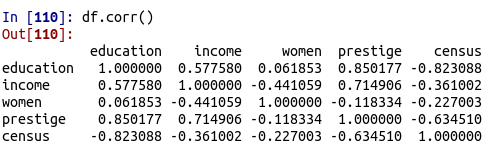You can see that there are several strong relationships. For our purposes, we will look at the relationship between education and income.

The seaborn library is rather easy to use for making visuals. To make a plot you can use the .lmplot() function. Below is a basic scatterplot of our data.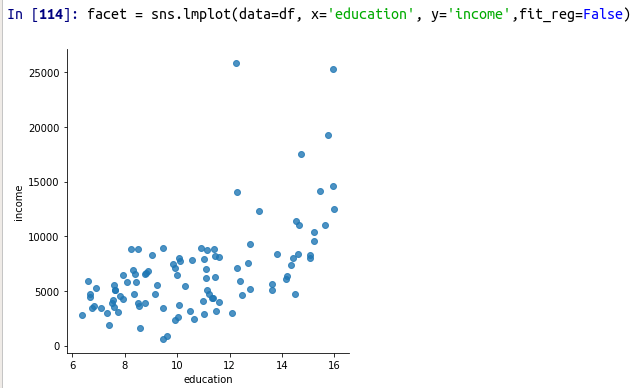The code should be self-explanatory. THe only thing that might be unknown is the fit_reg argument. This is set to False so that the function does not make a regression line. Below is the same visual but this time with the regression line.

`facet = sns.lmplot(data=df, x='education', y='income',fit_reg=True)`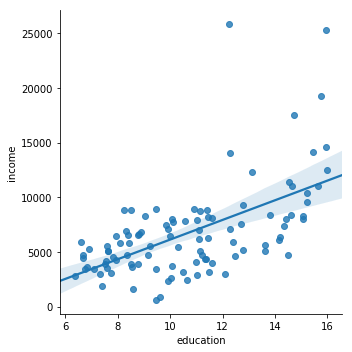It is also possible to add a third variable to our plot. One of the more common ways is through including a categorical variable. Therefore, we will look at job type and see what the relationship is. To do this we use the same .lmplot.() function but include several additional arguments. These include the hue and the indication of a legend. Below is the code and output.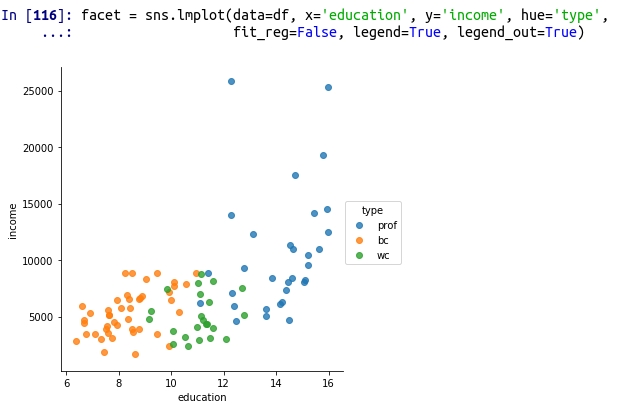You can clearly see that type separates education and income. A look at the boxplots for these variables confirms this.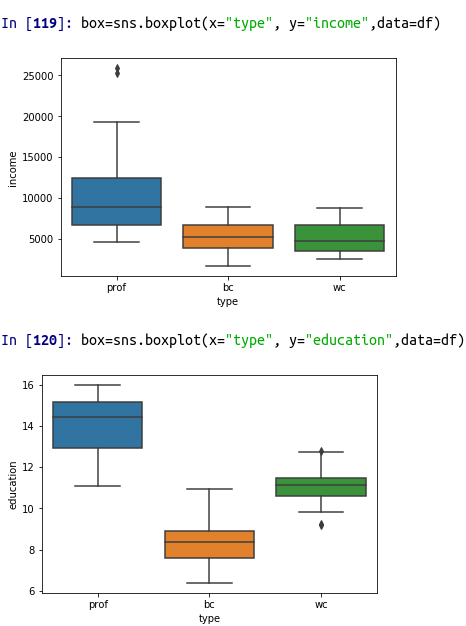As you can see, we can conclude that job type influences both education and income in this example.

Conclusion

This post focused primarily on making scatterplots with the seaborn package. Scatterplots are a tool that all data analyst should be familiar with as it can be used to communicate information to people who must make decisions.# Data Visualization in Python

In this post, we will look at how to set up various features of a graph in Python. The fine tune tweaks that can be made when creating a data visualization can be enhanced the communication of results with an audience. This will all be done using the matplotlib module available for python. Our objectives are as follows

• Make a graph with  two lines
• Set the tick marks
• Change the linewidth
• Change the line color
• Change the shape of the line
• Add a label to each axes
• Annotate the graph
• Add a legend and titleWe will use two variables from the “toothpaste” dataset from the pydataset module for this demonstration. Below is some initial code.

```from pydataset import data
import matplotlib.pyplot as plt
DF = data('toothpaste')```

Make Graph with Two Lines

To make a plot you use the .plot() function. Inside the parentheses you out the dataframe and variable you want. If you want more than one line or graph you use the .plot() function several times. Below is the code for making a graph with two line plots using variables from the toothpaste dataset.

```plt.plot(DF['meanA'])
plt.plot(DF['sdA'])```To get the graph above you must run both lines of code simultaneously. Otherwise, you will get two separate graphs.

Set Tick Marks

Setting the tick marks requires the use of the .axes() function. However, it is common to save this function in a variable called axes as a handle. This makes coding easier. Once this is done you can use the .set_xticks() function for the x-axes and .set_yticks() for the y axes. In our example below, we are setting the tick marks for the odd numbers only. Below is the code.

```ax=plt.axes()
ax.set_xticks([1,3,5,7,9])
ax.set_yticks([1,3,5,7,9])
plt.plot(DF['meanA'])
plt.plot(DF['sdA'])```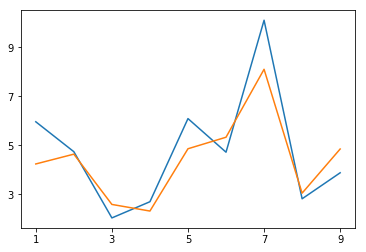Changing the Line Type

It is also possible to change the line type and width. There are several options for the line type. The important thing here is to put this information after the data you want to plot inside the code. Line width is changed with an argument that has the same name. Below is the code and visual

```ax=plt.axes()
ax.set_xticks([1,3,5,7,9])
ax.set_yticks([1,3,5,7,9])
plt.plot(DF['meanA'],'--',linewidth=3)
plt.plot(DF['sdA'],':',linewidth=3)```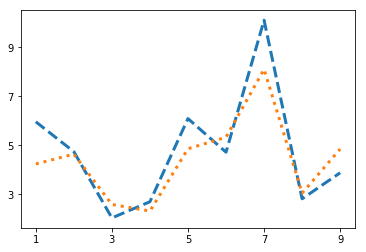Changing the Line Color

It is also possible to change the line color. There are several options available. The important thing is that the argument for the line color goes inside the same parentheses as the line type. Below is the code. r means red and k means black.

```ax=plt.axes()
ax.set_xticks([1,3,5,7,9])
ax.set_yticks([1,3,5,7,9])
plt.plot(DF['meanA'],'r--',linewidth=3)
plt.plot(DF['sdA'],'k:',linewidth=3)```Change the Point Type

Changing the point type requires more code inside the same quotation marks where the line color and line type are. Again there are several choices here. The code is below

```ax=plt.axes()
ax.set_xticks([1,3,5,7,9])
ax.set_yticks([1,3,5,7,9])
plt.plot(DF['meanA'],'or--',linewidth=3)
plt.plot(DF['sdA'],'Dk:',linewidth=3)```Add X and Y Labels

Adding LAbels is simple. You just use the .xlabel() function or .ylabel() function. Inside the parentheses, you put the text you want in quotation marks. Below is the code.

```ax=plt.axes()
ax.set_xticks([1,3,5,7,9])
ax.set_yticks([1,3,5,7,9])
plt.xlabel('X Example')
plt.ylabel('Y Example')
plt.plot(DF['meanA'],'or--',linewidth=3)
plt.plot(DF['sdA'],'Dk:',linewidth=3)```Adding Annotation, Legend, and Title

Annotation allows you to write text directly inside the plot wherever you want. This involves the use of the .annotate function. Inside this function, you must indicate the location of the text and the actual text you want added to the plot. For our example, we will add the word ‘python’ to the plot for fun.

The .legend() function allows you to give a description of the line types that you have included. Lastly, the .title() function allows you to add a title. Below is the code.

```ax=plt.axes()
ax.set_xticks([1,3,5,7,9])
ax.set_yticks([1,3,5,7,9])
plt.xlabel('X Example')
plt.ylabel('Y Example')
plt.annotate(xy=[3,4],text='Python')
plt.plot(DF['meanA'],'or--',linewidth=3)
plt.plot(DF['sdA'],'Dk:',linewidth=3)
plt.legend(['1st','2nd'])
plt.title("Plot Example")```Conclusion

Now you have a practical understanding of how you can communicate information visually with matplotlib in python. This is barely scratching the surface in terms of the potential that is available.# Random Forest Regression in Python

Random forest is simply the making of dozens if not thousands of decision trees. The decision each tree makes about an example are then tallied for the purpose of voting with the classification that receives the most votes winning. For regression, the results of the trees are averaged in  order to give the most accurate results

In this post, we will use the cancer dataset from the pydataset module to predict the age of people. Below is some initial code.

```import pandas as pd
import numpy as np
from pydataset import data
from sklearn.model_selection import train_test_split
from sklearn.model_selection import cross_val_score
from sklearn.ensemble import RandomForestRegressor
from sklearn.metrics import mean_squared_error```

We can load our dataset as df, drop all NAs, and create our dataset that contains the independent variables and a separate dataset that includes the dependent variable of age. The code is below

```df = data('cancer')
df=df.dropna()
X=df[['time','status',"sex","ph.ecog",'ph.karno','pat.karno','meal.cal','wt.loss']]
y=df['age']```

Next, we need to set up our train and test sets using a 70/30 split. After that, we set up our model using the RandomForestRegressor function. n_estimators is the number of trees we want to create and the random_state argument is for supporting reproducibility. The code is below

```x_train, x_test, y_train, y_test = train_test_split(X, y, test_size=0.3, random_state=0)
h=RandomForestRegressor(n_estimators=100,random_state=1)```

We can now run our model and test it. Running the model requires the .fit() function and testing involves the .predict() function. The results of the test are found using the mean_squared_error() function.

```h.fit(x_train,y_train)
y_pred=h.predict(x_test)
mean_squared_error(y_test,y_pred)
71.75780196078432
```

The MSE of 71.75 is only useful for model comparison and has little meaning by its self. Another way to assess the model is by determining variable importance. This helps you to determine in a descriptive way the strongest variables for the regression model. The code is below followed by the plot of the variables.

```model_ranks=pd.Series(h.feature_importances_,index=x_train.columns,name="Importance").sort_values(ascending=True,inplace=False)
ax=model_ranks.plot(kind='barh')```As you can see, the strongest predictors of age include calories per meal, weight loss, and time sick. Sex and whether the person is censored or dead make a smaller difference. This makes sense as younger people eat more and probably lose more weight because they are heavier initially when dealing with cancer.

Conclusison

This post provided an example of the use of regression with random forest. Through the use of ensemble voting, you can improve the accuracy of your models. This is a distinct power that is not available with other machine learning algorithm.# Bagging Classification with Python

Bootstrap aggregation aka bagging is a technique used in machine learning that relies on resampling from the sample and running multiple models from the different samples. The mean or some other value is calculated from the results of each model. For example, if you are using Decisions trees, bagging would have you run the model several times with several different subsamples to help deal with variance in statistics.Bagging is an excellent tool for algorithms that are considered weaker or more susceptible to variances such as decision trees or KNN. In this post, we will use bagging to develop a model that determines whether or not people voted using the turnout dataset. These results will then be compared to a model that was developed in a traditional way.

We will use the turnout dataset available in the pydataset module. Below is some initial code.

```from pydataset import data
import numpy as np
import pandas as pd
from sklearn.model_selection import train_test_split
from sklearn.ensemble import BaggingClassifier
from sklearn.neighbors import KNeighborsClassifier
from sklearn.model_selection import cross_val_score
from sklearn.metrics import classification_report```

We will load our dataset. Then we will separate the independnet and dependent variables from each other and create our train and test sets. The code is below.

```df=data("turnout")
X=df[['age','educate','income',]]
y=df['vote']
X_train,X_test,y_train,y_test=train_test_split(X,y,test_size=.3,random_state=0)```

We can now prepare to run our model. We need to first set up the bagging function. There are several arguments that need to be set. The max_samples argument determines the largest amount of the dataset to use in resampling. The max_features argument is the max number of features to use in a sample. Lastly, the n_estimators is for determining the number of subsamples to draw. The code is as follows

` h=BaggingClassifier(KNeighborsClassifier(n_neighbors=7),max_samples=0.7,max_features=0.7,n_estimators=1000)`

Basically, what we told python was to use up to 70% of the samples, 70% of the features, and make 100 different KNN models that use seven neighbors to classify. Now we run the model with the fit function, make a prediction with the predict function, and check the accuracy with the classificarion_reoirts function.

```h.fit(X_train,y_train)
y_pred=h.predict(X_test)
print(classification_report(y_test,y_pred))```This looks oka below are the results when you do a traditional model without bagging

```X_train,X_test,y_train,y_test=train_test_split(X,y,test_size=.3,random_state=0)
clf=KNeighborsClassifier(7)
clf.fit(X_train,y_train)
y_pred=clf.predict(X_test)
print(classification_report(y_test,y_pred))```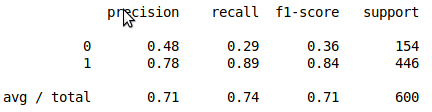The improvement is not much. However, this depends on the purpose and scale of your project. A small improvement can mean millions in the reight context such as for large companies such as Google who deal with billions of people per day.

Conclusion

This post provides an example of the use of bagging in the context of classification. Bagging provides a why to improve your model through the use of resampling.# K Nearest Neighbor Classification with Python

K Nearest Neighbor uses the idea of proximity to predict class. What this means is that with KNN Python will look at K neighbors to determine what the unknown examples class should be. It is your job to determine the K or number of neighbors that should be used to determine the unlabeled examples class.KNN is great for a small dataset. However, it normally does not scale well when the dataset gets larger and larger. As such, unless you have an exceptionally powerful computer KNN is probably not going to do well in a Big Data context.

In this post, we will go through an example of the use of KNN with the turnout dataset from the pydataset module. We want to predict whether someone voted or not based on the independent variables. Below is some initial code.

```from pydataset import data
import pandas as pd
from sklearn.model_selection import train_test_split
from sklearn.neighbors import KNeighborsClassifier
from sklearn.metrics import classification_report```

We now need to load the data and separate the independent variables from the dependent variable by making two datasets.

```df=data("turnout")
X=df[['age','educate','income']]
y=df['vote']```

Next, we will make our train and test sets with a 70/30 split. The random.state is set to 0. This argument allows us to reproduce our model if we want. After this, we will run our model. We will set the K to 7 for our model  and run the model. This means that Python will look at the 7 closes examples to predict the value of an unknown example. below is the code

```X_train,X_test,y_train,y_test=train_test_split(X,y,test_size=.3,random_state=0)
clf=KNeighborsClassifier(7)
clf.fit(X_train,y_train)```

We can now predict with our model and see the results with the classification reports function.

```y_pred=clf.predict(X_test)
print(classification_report(y_test,y_pred))```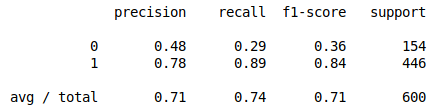The results are shown above. To determine the quality of the model relies more on domain knowledge. What we can say for now is that the model is better at classifying people who vote rather than people who do not vote.

Conclusion

This post shows you how to work with Python when using KNN. This algorithm is useful in using neighboring examples tot predict the class of an unknown example.# Naive Bayes with Python

Naive Bayes is a probabilistic classifier that is often employed when you have multiple or more than two classes in which you want to place your data. This algorithm is particularly used when you dealing with text classification with large datasets and many features.If you are more familiar with statistics you know that Bayes developed a method of probability that is highly influential today. In short, his system takes into conditional probability. In the case of naive Bayes,  the classifier assumes that the presence of a certain feature in a class is not related to the presence of any other feature. This assumption is why Naive Bayes is Naive.

For our purposes, we will use Naive Bayes to predict the type of insurance a person has in the DoctorAUS dataset in the pydataset module. Below is some initial code.

```from pydataset import data
import pandas as pd
from sklearn.naive_bayes import GaussianNB
from sklearn.model_selection import train_test_split
from sklearn.metrics import classification_report```

Next, we will load our dataset DoctorAUS. Then we will separate the independent variables that we will use from the dependent variable of insurance in two different datasets. If you want to know more about the dataset and the variables you can type data(“DoctorAUS”, show_doc=True)

```df=data("DoctorAUS")
y=df['insurance']```

Now, we will create our train and test datasets. We will do a 70/30 split. We will also use Gaussian Naive Bayes as our algorithm. This algorithm assumes the data is normally distributed. There are other algorithms available for Naive Bayes as well.  We will also create our model with the .fit function.

```X_train,X_test,y_train,y_test=train_test_split(X,y,test_size=.3,random_state=0)
clf=GaussianNB()
clf.fit(X_train,y_train)```

Finally, we will predict with our model and run the classification report to determine the success of the model.

```y_pred=clf.predict(X_test)
print(classification_report(y_test,y_pred))```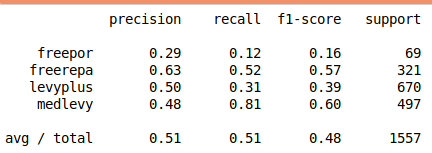You can see that our overall numbers are not that great. This means that the current algorithm is probably not the best choice for classification. Of course, there could other problems as well that need to be explored.

Conclusion

This post was simply a demonstration of how to conduct an analysis with Naive Bayes using Python. The process is not all that complicate and is similar to other algorithms that are used.# K Nearest Neighbor Regression with Python

K Nearest Neighbor Regression (KNN) works in much the same way as KNN for classification. The difference lies in the characteristics of the dependent variable. With classification KNN the dependent variable is categorical. WIth regression KNN the dependent variable is continuous. Both involve the use neighboring examples to predict the class or value of other examples.This post will provide an example of KNN regression using the turnout dataset from the pydataset module. Our purpose will be to predict the age of a voter through the use of other variables in the dataset. Below is some initial code.

```from pydataset import data
import pandas as pd
from sklearn.model_selection import train_test_split
from sklearn.neighbors import KNeighborsRegressor
from sklearn.metrics import mean_squared_error```

We now need to setup our data. We need to upload our actual dataset. Then we need to separate the independnet and dependent variables. Once this is done we need to create our train and test sets using the tarin test spli t funvtion. Below is the code to accmplouh each of these steps.

```df=data("turnout")
X=df[['age','income','vote']]
y=df['educate']
X_train,X_test,y_train,y_test=train_test_split(X,y,test_size=.3,random_state=0)```

We are now ready to train our model. We need to call the function we will use and determine the size of K, which will be 11 in our case. Then we need to train our model and then predict with it. Lastly, we will print out the mean squared error. This value is useful for comparing models but does not have much value by itself. The MSE is calculated by comparing the actual test set with the predicted test data. The code is below

```clf=KNeighborsRegressor(11)
clf.fit(X_train,y_train)
y_pred=clf.predict(X_test)
print(mean_squared_error(y_test,y_pred))```
`9.239`

If we were to continue with model development we may look for ways to improve our MAE through different nethods such as regular linear regression. However, for our purposes this is adequate.

Conclusison

This post provides an example of regression with KNN in Python. This tool is a practical and simple way to make numeric predictions that can be accurate at times.# Support Vector Machines Regression with Python

This post will provide an example of how to do regression with support vector machines SVM. SVM is a complex algorithm that allows for the development of non-linear models. This is particularly useful for messy data that does not have clear boundaries.The steps that we will use are listed below

1. Data preparation
2. Model Development

We will use two different kernels in our analysis. The LinearSVR kernel and SVR kernel. The difference between these two kernels has to do with slight changes in the calculations of the boundaries between classes.

Data Preparation

We are going to use the OFP dataset available in the pydataset module. This dataset was used previously for classification with SVM on this site. Our plan this time is that we want to predict family inc (famlinc), which is a continuous variable.  Below is some initial code.

```import numpy as np
import pandas as pd
from pydataset import data
from sklearn import svm
from sklearn import model_selection
from statsmodels.tools.eval_measures import mse```

We now need to load our dataset and remove any missing values.

```df=pd.DataFrame(data('OFP'))
df=df.dropna()```

AS in the previous post, we need to change the text variables into dummy variables and we also need to scale the data. The code below creates the dummy variables, removes variables that are not needed, and also scales the data.

```dummy=pd.get_dummies(df['black'])
df=pd.concat([df,dummy],axis=1)
df=df.rename(index=str, columns={"yes": "black_person"})
df=df.drop('no', axis=1)

dummy=pd.get_dummies(df['sex'])
df=pd.concat([df,dummy],axis=1)
df=df.rename(index=str, columns={"male": "Male"})
df=df.drop('female', axis=1)

dummy=pd.get_dummies(df['employed'])
df=pd.concat([df,dummy],axis=1)
df=df.rename(index=str, columns={"yes": "job"})
df=df.drop('no', axis=1)

dummy=pd.get_dummies(df['maried'])
df=pd.concat([df,dummy],axis=1)
df=df.rename(index=str, columns={"no": "single"})
df=df.drop('yes', axis=1)

dummy=pd.get_dummies(df['privins'])
df=pd.concat([df,dummy],axis=1)
df=df.rename(index=str, columns={"yes": "insured"})
df=df.drop('no', axis=1)
df=df.drop(['black','sex','maried','employed','privins','medicaid','region','hlth'],axis=1)
df = (df - df.min()) / (df.max() - df.min())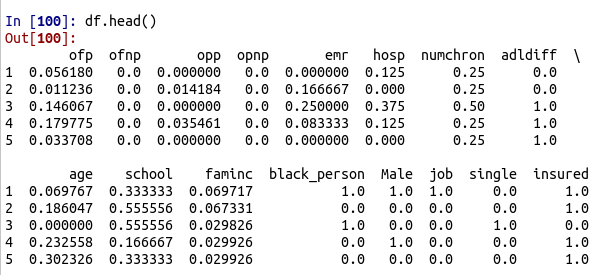We now need to set up our datasets. The X dataset will contain the independent variables while the y dataset will contain the dependent variable

```X=df[['ofp','ofnp','opp','opnp','emr','hosp','numchron','adldiff','age','school','single','black_person','Male','job','insured']]
y=df['faminc']```

We can now move to model development

Model Development

We now need to create our train and test sets for or X and y datasets. We will do a 70/30 split of the data. Below is the code

`X_train,X_test,y_train,y_test=model_selection.train_test_split(X,y,test_size=.3,random_state=1)`

Next, we will create our two models with the code below.

```h1=svm.SVR()
h2=svm.LinearSVR()```

We will now run our first model and assess the results. Our metric is the mean squared error. Generally, the lower the number the better.  We will use the .fit() function to train the model and the .predict() function for test the model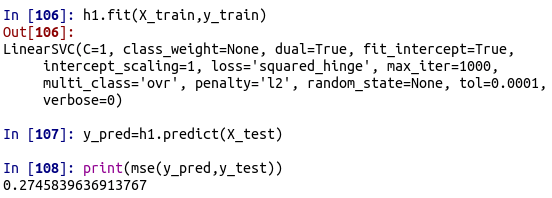The mse was 0.27. This number means nothing only and is only beneficial for comparison reasons. Therefore, the second model will be judged as better or worst only if the mse is lower than 0.27. Below are the results of the second model.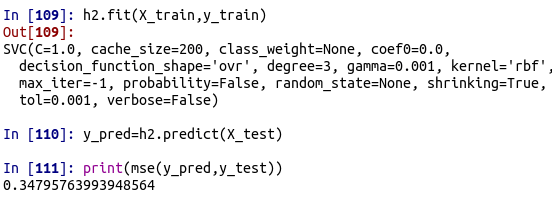We can see that the mse for our second model is 0.34 which is greater than the mse for the first model. This indicates that the first model is superior based on the current results and parameter settings.

Conclusion

This post provided an example of how to use SVM for regression.# Support Vector Machines Classification with Python

Support vector machines (SVM) is an algorithm used to fit non-linear models. The details are complex but to put it simply  SVM tries to create the largest boundaries possible between the various groups it identifies in the sample. The mathematics behind this is complex especially if you are unaware of what a vector is as defined in algebra.This post will provide an example of SVM using Python broken into the following steps.

1. Data preparation
2. Model Development

We will use two different kernels in our analysis. The linear kernel and he rbf kernel. The difference in terms of kernels has to do with how the boundaries between the different groups are made.

Data Preparation

We are going to use the OFP dataset available in the pydataset module. We want to predict if someone single or not. Below is some initial code.

```import numpy as np
import pandas as pd
from pydataset import data
from sklearn import svm
from sklearn.metrics import classification_report
from sklearn import model_selection```

We now need to load our dataset and remove any missing values.

```df=pd.DataFrame(data('OFP'))
df=df.dropna()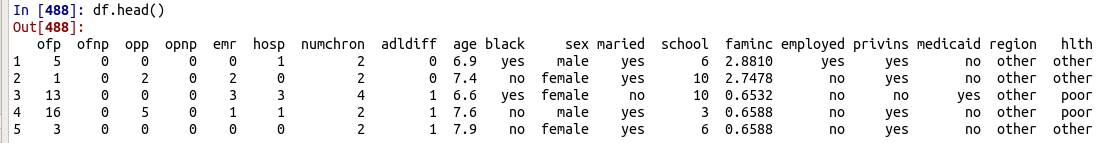Looking at the dataset we need to do something with the variables that have text. We will create dummy variables for all except region and hlth. The code is below.

```dummy=pd.get_dummies(df['black'])
df=pd.concat([df,dummy],axis=1)
df=df.rename(index=str, columns={"yes": "black_person"})
df=df.drop('no', axis=1)

dummy=pd.get_dummies(df['sex'])
df=pd.concat([df,dummy],axis=1)
df=df.rename(index=str, columns={"male": "Male"})
df=df.drop('female', axis=1)

dummy=pd.get_dummies(df['employed'])
df=pd.concat([df,dummy],axis=1)
df=df.rename(index=str, columns={"yes": "job"})
df=df.drop('no', axis=1)

dummy=pd.get_dummies(df['maried'])
df=pd.concat([df,dummy],axis=1)
df=df.rename(index=str, columns={"no": "single"})
df=df.drop('yes', axis=1)

dummy=pd.get_dummies(df['privins'])
df=pd.concat([df,dummy],axis=1)
df=df.rename(index=str, columns={"yes": "insured"})
df=df.drop('no', axis=1)```

For each variable, we did the following

1. Created a dummy in the dummy dataset
2. Combined the dummy variable with our df dataset
3. Renamed the dummy variable based on yes or no
4. Drop the other dummy variable from the dataset. Python creates two dummies instead of one.

If you look at the dataset now you will see a lot of variables that are not necessary. Below is the code to remove the information we do not need.

```df=df.drop(['black','sex','maried','employed','privins','medicaid','region','hlth'],axis=1)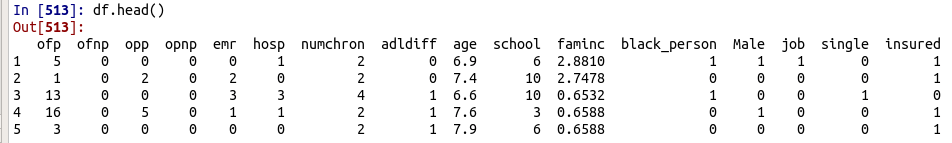This is much cleaner. Now we need to scale the data. This is because SVM is sensitive to scale. The code for doing this is below.

```df = (df - df.min()) / (df.max() - df.min())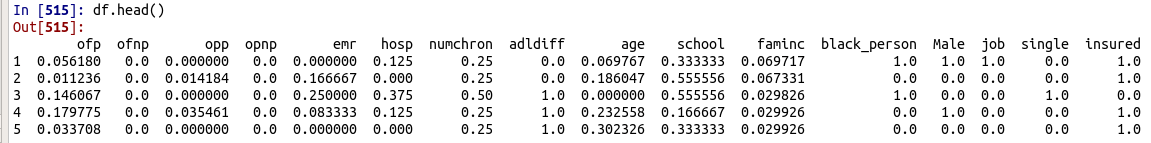We can now create our dataset with the independent variables and a separate dataset with our dependent variable. The code is as follows.

```X=df[['ofp','ofnp','opp','opnp','emr','hosp','numchron','adldiff','age','school','faminc','black_person','Male','job','insured']]
y=df['single']```

We can now move to model development

Model Development

We need to make our test and train sets first. We will use a 70/30 split.

`X_train,X_test,y_train,y_test=model_selection.train_test_split(X,y,test_size=.3,random_state=1)`

Now, we need to create the models or the hypothesis we want to test. We will create two hypotheses. The first model is using a linear kernel and the second is one using the rbf kernel. For each of these kernels, there are hyperparameters that need to be set which you will see in the code below.

```h1=svm.LinearSVC(C=1)
h2=svm.SVC(kernel='rbf',degree=3,gamma=0.001,C=1.0)```

The details about the hyperparameters are beyond the scope of this post. Below are the results for the first model.The overall accuracy is 73%. The crosstab() function provides a breakdown of the results and the classification_report() function provides other metrics related to classification. In this situation, 0 means not single or married while 1 means single. Below are the results for model 2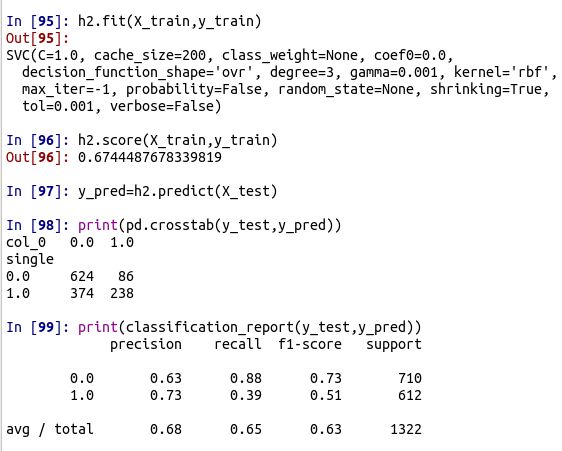You can see the results are similar with the first model having a slight edge. The second model really struggls with predicting people who are actually single. You can see thtat the recall in particular is really poor.

Conclusion

This post provided how to ob using SVM in python. How this algorithm works can be somewhat confusing. However, its use can be powerful if use appropriately.